# JP5034469B2 - Information processing apparatus, information processing method, and program - Google Patents

## Info

Publication number
JP5034469B2
JP5034469B2 JP2006331823A JP2006331823A JP5034469B2 JP 5034469 B2 JP5034469 B2 JP 5034469B2 JP 2006331823 A JP2006331823 A JP 2006331823A JP 2006331823 A JP2006331823 A JP 2006331823A JP 5034469 B2 JP5034469 B2 JP 5034469B2
Authority
JP
Japan
Prior art keywords
state
learning
separation matrix
separation
Prior art date
Legal status (The legal status is an assumption and is not a legal conclusion. Google has not performed a legal analysis and makes no representation as to the accuracy of the status listed.)
Expired - Fee Related
Application number
JP2006331823A
Other languages
Japanese (ja)
Other versions
JP2008147920A (en
Inventor

Original Assignee
ソニー株式会社
Priority date (The priority date is an assumption and is not a legal conclusion. Google has not performed a legal analysis and makes no representation as to the accuracy of the date listed.)
Filing date
Publication date
Application filed by ソニー株式会社 filed Critical ソニー株式会社
Priority to JP2006331823A priority Critical patent/JP5034469B2/en
Publication of JP2008147920A publication Critical patent/JP2008147920A/en
Application granted granted Critical
Publication of JP5034469B2 publication Critical patent/JP5034469B2/en
Application status is Expired - Fee Related legal-status Critical
Anticipated expiration legal-status Critical

• 238000004458 analytical methods Methods 0 abstract 1

## Images

•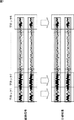•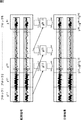•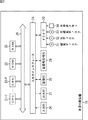•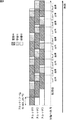•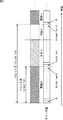•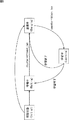••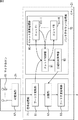•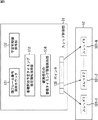•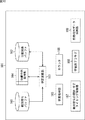•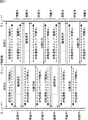•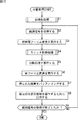••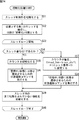•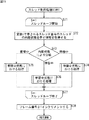•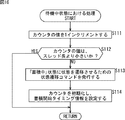••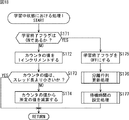••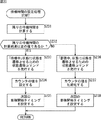••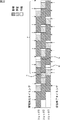•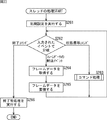•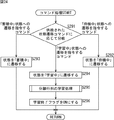••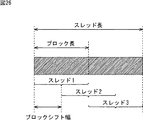•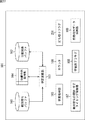•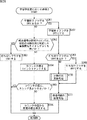•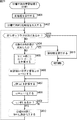•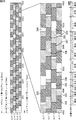## Abstract

<P>PROBLEM TO BE SOLVED: To eliminate a tradeoff between a block length of an ICA (Independent Component Analysis) in a block unit and an update interval of a separation matrix. <P>SOLUTION: In respective thread 1 to thread 3, observation signals of designated time are stored in a buffer in the state of "being storing". After the designated time elapses, the state shifts to being learning, a learning processing loop is executed until the separation matrix W is converged (or the number of fixed times), and a separation matrix corresponding to observation signals stored in the state of being storing is calculated. In the state of being standby, the observation signals are not stored and learning is not executed, but they are made standby only for the designated time. Time for maintaining the stat of being standby is determined by time required for learning. Each of the thread 1 to the thread 3 is shifted only by a predetermined block shift with (Block_shift) and started. For example, a half of block length may be defined as block shift width. The invention is applicable to a sound source dividing device. <P>COPYRIGHT: (C)2008,JPO&amp;INPIT

## Description

The present invention relates to an information processing device, an information processing method, and a program, and more particularly, to an information processing device, an information processing method, and a program that are suitable for performing independent component analysis.

Independent component analysis (ICA) is a technique for decomposing a plurality of observed signals into statistically independent components.

A conventional technique for performing ICA in real time will be described below. Note that the real-time processing here means that an observation signal that is continuously input is decomposed (separated) into independent components with a small delay and is output continuously.

ICA is formulated as follows.

As shown in Equation (1), let x 1 (t), ..., x n (t) be a plurality of observed signals at a certain time t, and let x (t) be a vector with these components as components. .

Then, the result of multiplying the matrix in x (t) is y (t) shown in Equation (2).

And y (t) shown in Formula (2) can also be expressed as a vector having a plurality of components, as shown in Formula (3).

ICA is to obtain the matrix W shown in equation (3) such that y 1 (t), ..., y n (t), which are components of y (t), are statistically independent. Yes, such a matrix is called a separation matrix.

In this description, x (t) and y (t) are assumed to be signals at time t in the time domain, but instead, in the t th frame of the signal (spectrogram) in the time frequency domain. You can think of it as a signal.

Various algorithms are known as algorithms for obtaining the separation matrix W, but they are mainly classified into two algorithms, an online algorithm and a batch algorithm.

The online algorithm is a method of updating the separation matrix every time one set of observation signals is supplied. Hereinafter, ICA using an online algorithm is also simply referred to as online processing.

The online processing is executed by repeatedly executing the following equations (4) to (7).

In equation (5), φ is a function called a score function or activation function, and in equation (6), η is a small positive constant called a learning coefficient. Each time Expressions (4) to (7) are executed, one observation signal vector x (t) is supplied, and the separation matrix W is updated each time.

Since this method does not include a loop and does not require accumulation of observation signals, it can be applied to real-time processing as it is. However, this method has the disadvantage that a large number of samples are required before the separation matrix W converges. That is, when the sound source separation system is constructed using the online processing shown in the equations (4) to (7), the output itself is generated without delay, but until the result is sufficiently separated. Takes some time from startup.

On the other hand, the batch algorithm accumulates observation signals for a certain amount of time (for example, for one utterance), and repeats the update formula for the separation matrix W until the separation matrix W converges (or a fixed number of times) for that data. This is the method to apply.

Hereinafter, this repeated application will be referred to as learning. Then, applying the separation matrix W to the observation signal X to calculate the separation result y = Wx is referred to as separation. In addition, ICA using a batch algorithm is simply referred to as batch processing. In y = Wx, x and y represent observation signals or separation results at all times, respectively. That is, assuming that all times are T, x = [x (1),... X (T)] and y = [y (1),... Y (T)].

The online processing is executed by repeatedly executing the following equations (8) to (10).

By repeatedly executing Expressions (8) to (10), W and y (t) converge to a certain value. In Equation (9), E t [] means averaging in the time direction, that is, taking the average among accumulated samples.

Since batch processing requires time for data accumulation and a learning loop, it is necessary to devise in order to apply it to real-time processing. For example, there is a technique that can be applied to real-time processing by performing batch processing in block units (hereinafter simply referred to as block processing) (see, for example, Non-Patent Document 1).

"Blind speech separation of moving speakers in real reverberant environment," A. Koutras, E. Dermatas, and G. Kokkinakis, in Proc. Of ICASSP'00, 2000, pp. 1133.1136.

There is also a technique for performing ICA using block processing (see, for example, Patent Document 1).

JP 2005-227512 A

Block processing will be described with reference to FIG.

In the block processing, the observation signal (here, for example, sound signals collected by a plurality of microphones) is divided into blocks of a predetermined time (for example, about 1 to 3 seconds), and batch processing is performed for each block. That is, when an observation signal for one block is input, learning is performed on the observation signal for one block, and a separation result for one block is output.

In this way, it is possible to output the separation results continuously without interrupting the input. However, a delay from input to output also occurs in block processing. The delay that occurs is the sum of the block length and the time required for learning. For example, if the block length in the block processing is 1 second and the time required for learning is 0.5 seconds, the output delay with respect to the input is 1.5 seconds.

As described above, since delay time occurs in block processing, it has been proposed to reduce the delay by performing separation using the separation matrix obtained in the previous block (for example, non-patent literature). 2 or non-patent document 3).

"Robust Real-Time Blind Source Separation for Moving Speakers in a Room," R. Mukai, H. Sawada, S. Araki, S. Makino, in Proc. Of ICASSP2003, Vol. 5, pp.469-472, Apr. 2003

"Low-delay real-time blind separation of moving sound sources" Mukai, Sawada, Araki, Makino. Oncology, pp. 779.780, Mar. 2003.

Block processing that performs separation using the separation matrix obtained in the previous block is hereinafter referred to as improved block processing.

With reference to FIG. 2, the outline of the improved block processing will be described.

Also in the improved block processing, the observation signal is divided into blocks of a fixed time (for example, about 1 to 3 seconds), and batch processing is performed for each block.

When the observation signal X (t) belonging to the kth block X [k] from the top is separated, the separation matrix W [k] corresponding to the kth block X [k] is input when the observation signal is input . Is, of course, not yet sought after. So, if the learning time is shorter than the block length, using the fact that W [k-2] is already obtained when X (t) is observed, instead of the separation matrix W [k] , It is assumed that the separation result Y = W × X is calculated using the separation matrix W [k−2] learned from the previous block. That is, in the improved block processing, to separate the observation signal X (t) at a certain time and obtain the separation result Y (t), Y (t) = W [k-2] × X (t) Calculated.

As a result, it is possible to output separation results continuously without interrupting input, and separation can be performed with a small delay. Note that the time required for the separation process, that is, the calculation of Y (t) = W [k−2] × X (t) is shorter than the accumulation and learning of observation signals. However, strictly speaking, ICA in the time-frequency domain requires short-time Fourier transform and inverse transform before and after ICA, so even with the improved block processing, the delay by the number of taps of Fourier transform Arise.

For example, when a sound signal collected by a plurality of microphones is decomposed into components that are statistically independent using independent component analysis (ICA), the separation matrix W is calculated as follows. It reflects the spatial transfer characteristics between the two. Therefore, when the transfer characteristic does not change, that is, when neither the sound source nor the microphone moves, even if the improved block processing is performed, that is, the block used to obtain the separation matrix W and the block to which the separation matrix W is applied Even if they are different, a sufficiently separated result can be obtained.

On the other hand, if the microphone or sound source is moving, a block X [k-2] used in the calculation of the separating matrix, for spatial transfer characteristics between the block X [k] applying the separating matrix is changed , instead of the separation matrix W [k], the separation results obtained by using the learned from two previous block the separation matrix W [k-2] Y ( t) = W [k-2] × X (t ) Is not a complete separation result due to the following delay. That is, when the microphone or the sound source moves, it is impossible to obtain an accurate separation result by using the improved block processing.

Further, in the improved block processing, a trade-off occurs between separation performance and follow-up delay. Specifically, when the sound source or the microphone does not move, the performance of separation is improved by learning using a long-time observation signal (that is, by increasing the length of the block). On the other hand, when the sound source or the microphone moves, the tracking delay increases if the block is long.

In addition, in order to use the separation matrix W [k-2] obtained from the previous block, W [k-2] has already been obtained when the first observation signal of W [k] is input. Need to be. That is, the time required for learning must be shorter than the block length. However, the learning time is not always constant. Further, for example, in an information processing apparatus such as a personal computer equipped with a multitasking OS, when the improved block processing described above is executable (implemented), learning is required when only the improved block processing is considered. Even if the time is shorter than the block length on average, there is a possibility that the processor is temporarily heavily loaded due to the influence of other processes during the learning process, and the learning time may exceed the block length.

As described above, when the time required for learning cannot be guaranteed to be shorter than the block length, the above-described improved block processing cannot obtain a correct separation result.

In other words, when executing real-time processing in a sound source separation system using ICA, the conventional technology eliminates the trade-off between block length and tracking delay, updates the block length and separation matrix update interval, and learning time. There was a request to set up freely. Note that the real-time processing here means that an observation signal that is continuously input is decomposed into independent components with a small delay and is output continuously.

The present invention has been made in view of such a situation, and when performing sound source separation in real time using independent component analysis (ICA), it is decomposed into independent components with a small delay, It makes it possible to output it continuously.

An information processing apparatus according to an aspect of the present invention is an information processing apparatus that performs independent component analysis, and uses an acquisition unit that acquires a signal and the signal acquired by the acquisition unit to perform the independent component analysis. Learning means for obtaining a separation matrix by learning; and separation means for generating a separation result by applying the separation matrix obtained by the learning means to the signal obtained by the obtaining means; The means includes at least two states of a first state in which the signal is accumulated and a second state in which the separation matrix is calculated by executing batch processing based on the independent component analysis using the accumulated signal. a, comprising a plurality of arithmetic means for obtaining by learning the separating matrix, and an arithmetic control unit for controlling a plurality of said calculation means, said calculation means, said first state Oyo Nor any state of the second state, further comprising a third state of waiting for processing, the arithmetic control unit controls the time of the third state in the operation means, a plurality of The plurality of calculation means are controlled so that the start timing of the first state in each of the calculation means is substantially evenly shifted in a period shorter than the block length, which is a unit period for learning the separation matrix.

The calculation control means controls a plurality of the calculation means so that timings of at least a part of the first state and the second state overlap in any of the plurality of calculation means. can do. Thereby, for example, the trade-off between the sound source separation performance and the sound source tracking performance, which the conventional block processing has, can be solved.

The calculation means may further include a third state that waits for processing, not the first state or the second state, and the calculation control means includes the calculation state. By controlling the time of the third state in the means, the plurality of computing means can be controlled so that the start timing of the first state in each of the plurality of computing means is shifted. .

The arithmetic control means includes a plurality of control units that control the time of the third state in the arithmetic means so that the start timing of the first state in each of the plurality of arithmetic means is substantially evenly shifted. The calculation means can be controlled.

The arithmetic control means controls the time of the third state in the arithmetic means so that at least a part of the timings of the first state and the second state can be any of the plurality of arithmetic means. A plurality of the arithmetic means can be controlled so as to overlap each other.

In the calculation means, the first state can be continued for a predetermined fixed period, and the calculation control means has a timing at which the first state starts in any of the calculation means. A plurality of the calculation means can be controlled such that the interval is shorter than the predetermined period during which the first state is continued.

In the calculation control unit, when the calculation unit of any of the plurality of calculation units calculates the separation matrix by executing batch processing based on the independent component analysis, the calculated separation matrix is Whether the signal accumulation interval accumulated in the first state used for obtaining is newer than the signal accumulation interval used for obtaining the separation matrix already calculated by the other arithmetic means. If it is determined that it is not new, the calculated separation matrix can be discarded.

In the calculation control unit, when the calculation unit of any of the plurality of calculation units calculates the separation matrix by executing batch processing based on the independent component analysis, the calculated separation matrix is So that the batch processing by the other arithmetic means being executed using the signal accumulated in the section earlier than the accumulation period of the signal accumulated in the first state used for obtaining is canceled, The other calculation means can be controlled.

The arithmetic control means includes a plurality of the separation matrices that are most recently obtained in any of the plurality of arithmetic means as the initial value of the separation matrix used for learning that the arithmetic means obtains the separation matrix. The calculation means can be controlled.

In the arithmetic control means, as the initial value of the separation matrix used for learning in which the arithmetic means obtains the separation matrix, the separation matrix obtained most recently in any of the plurality of arithmetic means and previously obtained A plurality of the calculation means can be controlled so that values obtained by calculation using a separation matrix obtained by past learning are used.

In the calculation control means, the plurality of calculation means hold the signal in the same signal holding means in the first state, and the signal held by the signal holding means in the second state. As used, a plurality of the calculating means can be controlled. This can be said, for example, that a plurality of calculation means share the signal holding means.

The computing means is caused to obtain the separation matrix used for independent component analysis by the signal processing means by learning using a part of the signals obtained by the obtaining means in the first state. Can be.

An information processing method according to an aspect of the present invention is an information processing method for an information processing apparatus that performs independent component analysis, and acquires a signal, stores the acquired signal, and the stored first state. In a plurality of calculation units having at least two states of a second state in which the separation matrix is calculated by performing batch processing based on the independent component analysis using a signal, and obtaining the separation matrix by learning Controlling a process for obtaining a separation matrix to be used for processing by learning, and applying the separation matrix to the acquired signal to generate a separation result, wherein the computing unit includes the first state and nor any state of the second state, further comprising a third state of waiting for processing, by controlling the time of the third state in the operation portion, a plurality of the Start timing of the first state in each of the calculation unit, said to be shifted substantially uniformly in a shorter period of time than the block length which is a unit period for learning of the separating matrix, the process of the learning by the plurality of the arithmetic unit Is controlled.

A program according to one aspect of the present invention is a program for causing a computer to execute a process of performing independent component analysis, the first state of controlling acquisition of a signal and storing the signal of which acquisition is controlled. A plurality of operations that have at least two states of a second state in which the separation matrix is calculated by executing batch processing based on the independent component analysis using the accumulated signal and that obtain the separation matrix by learning And a step of generating a separation result by controlling a process for obtaining a separation matrix used for the separation process by learning and applying the separation matrix to the acquired signal . It is not in any of the state 1 and the second state, but further has a third state waiting for processing, and the time of the third state in the arithmetic unit is calculated. By Gosuru, start timing of the first state in each of the plurality of the arithmetic unit, wherein a unit period performing learning of the separating matrix to be shifted substantially uniformly in a shorter period than the block length, a plurality of A computer is caused to execute a process for controlling the learning process by the arithmetic unit.

In one aspect of the present invention, a signal is acquired, and a first state in which the acquired signal is stored and a batch process based on independent component analysis is performed using the stored signal to calculate a separation matrix. A plurality of arithmetic units that have at least two states of 2 and obtain a separation matrix by learning are used, a separation matrix used for separation processing is obtained by learning, and the separation matrix is applied to the acquired signal. As a result, a separation result is generated. The computing unit is not in either the first state or the second state, but has a third state waiting for processing, and the time of the third state in the computing unit is controlled. , the start timing of the first state in each of the plurality of operation portions, learning is controlled by a plurality of operation portions so as to be offset substantially uniformly in a shorter period of time than the block length which is a unit period for learning of the separating matrix .

The sound source separation device may be an independent device or a block that performs sound source separation processing of the information processing device.

According to one aspect of the present invention, independent component analysis can be performed, and in particular, the learning process is performed in parallel with a plurality of threads, and the timing of the state transition of each thread is shifted. Independent component analysis can be performed well, and it can be decomposed into independent components with a small delay and output continuously.

Embodiments of the present invention will be described below. Correspondences between constituent elements of the present invention and the embodiments described in the specification or the drawings are exemplified as follows. This description is intended to confirm that the embodiments supporting the present invention are described in the specification or the drawings. Therefore, even if there is an embodiment which is described in the specification or the drawings but is not described here as an embodiment corresponding to the constituent elements of the present invention, that is not the case. It does not mean that the form does not correspond to the constituent requirements. Conversely, even if an embodiment is described here as corresponding to a configuration requirement, that means that the embodiment does not correspond to a configuration requirement other than the configuration requirement. It's not something to do.

An information processing apparatus according to an aspect of the present invention is an information processing apparatus that performs independent component analysis, and includes an acquisition unit that acquires a signal (for example, a sound signal acquisition unit 29) and the signal acquired by the acquisition unit. And learning means (for example, the background processing unit 72 in FIG. 8) for obtaining a separation matrix by learning of the independent component analysis, and the signal obtained by the obtaining means to the signal obtained by the learning means. A separation unit that generates a separation result by applying a separation matrix (for example, the signal processing unit 71 in FIG. 8), and the learning unit stores a first state (for example, “accumulating in progress”). ”State) and a second state (for example,“ learning ”state) in which the separation matrix is calculated by executing batch processing based on the independent component analysis using the accumulated signal. A plurality of arithmetic means (for example, threads 101-1 to 101-N in FIG. 8) having at least two states and obtaining the separation matrix by learning, and an arithmetic control means (for example, controlling the plurality of arithmetic means) , And the calculation means is in either of the first state and the second state, and is in a third state (for example, “waiting”). ”State), and the calculation control unit controls the time of the third state in the calculation unit, so that the start timing of the first state in each of the plurality of calculation units is The plurality of arithmetic means are controlled so as to be substantially evenly shifted in a period shorter than the block length which is a unit period for learning the separation matrix.

The arithmetic control unit is configured such that the arithmetic unit is used as an initial value of a separation matrix used for learning to obtain the separation matrix, and the separation matrix most recently obtained in any of the plurality of arithmetic units and a past obtained previously. And a value obtained by calculation using a separation matrix obtained by learning (for example, W_init = (W [k] + W [k-1] +... + W [kN] ) / (N + 1) or aW + (1− a) W_init, where W [k] is a k-th separation matrix obtained after the system is started), and the plurality of arithmetic means can be controlled. .

An information processing method according to one aspect of the present invention is an information processing method of an information processing apparatus that performs independent component analysis, acquires a signal (for example, the process of step S2 in FIG. 12), and uses the acquired signal. A second state (e.g., computing the separation matrix by performing a batch process based on the independent component analysis using the accumulated first state (e.g., "accumulating" state) and the accumulated signal (e.g., A separation matrix used for separation processing in a plurality of arithmetic units (for example, threads 101-1 to 101-N in FIG. 8) that have at least two states of “learning” state and obtain the separation matrix by learning. Is controlled by learning (for example, the process of step S4 in FIG. 12), and the separation matrix is applied to the acquired signal to generate a separation result (for example, FIG. 12). Processing in step S5) includes the step, the arithmetic unit is not in any state of the first state and the second state, a third state of waiting for processing (e.g., the "waiting" state) A unit period in which the start timing of the first state in each of the plurality of computing units learns the separation matrix by controlling the time of the third state in the computing unit. The learning process by the plurality of arithmetic units is controlled so as to be substantially evenly shifted in a period shorter than the block length.

A program according to one aspect of the present invention is a program for causing a computer to execute processing for executing independent component analysis, and controls acquisition of signals (for example, processing in step S2 in FIG. 12), and acquisition is controlled. A second state in which the separation matrix is calculated by executing batch processing based on the independent component analysis using the first state in which the signal is accumulated (for example, the “accumulating” state) and the accumulated signal. In a plurality of arithmetic units (for example, threads 101-1 to 101-N in FIG. 8) having at least two states (for example, “learning” state) and obtaining the separation matrix by learning, separation processing is performed. Control processing for obtaining a separation matrix to be used by learning (for example, processing in step S4 of FIG. 12), and applying the separation matrix to the acquired signal Generating a result (for example, step S5 in FIG. 12) includes the step, the arithmetic unit is not in any state of the first state and the second state, a third state of waiting for processing (For example, a “standby” state), and by controlling the time of the third state in the arithmetic unit, the start timing of the first state in each of the arithmetic units is , Causing the computer to execute a process for controlling the learning process by the plurality of arithmetic units so as to shift substantially evenly in a period shorter than a block length that is a unit period for learning the separation matrix.

Hereinafter, embodiments of the present invention will be described with reference to the drawings.

FIG. 3 shows a block diagram of the sound source separation device 11.

CPUs (Central Processing Units) 21-1 to 21 -P are multiprocessors, and are loaded from a program stored in a ROM (Read Only Memory) 22 or a RAM (Random Access Memory) 23 from a storage unit 28. Various processes are executed according to the program. The RAM 23 also appropriately stores data necessary for the CPU 21 to execute various processes.

The CPU 21, ROM 22, and RAM 23 are connected to each other via a bus 24. An input / output interface 24 is also connected to the bus 24.

The input / output interface 24 includes an input unit 26 including a keyboard and a mouse, an output unit 27 including a display and a speaker, a storage unit 28 including a hard disk, and a sound signal acquisition unit 29 that acquires a sound signal. It is connected. The sound signal acquisition unit 29 includes, for example, a plurality of microphones and an AD conversion unit, and converts a sound signal collected by the plurality of microphones into a digital signal by processing of the AD conversion unit.

A drive 30 is connected to the input / output interface 24 as necessary, and a magnetic disk 31, an optical disk 32, a magneto-optical disk 33, a semiconductor memory 34, or the like is appropriately mounted, and a computer program read from them is loaded. These are installed in the storage unit 28 as necessary.

The CPU 21 performs independent component analysis (ICA) on the sound signal collected by the plurality of microphones acquired by the sound signal acquisition unit 29 and converted into a digital signal.

When executing the ICA, the CPU 21 uses a unit of processing called a thread to perform sequential processing, that is, processing for outputting a separation signal from an observation signal in substantially real time.

The CPU 21 operates a plurality of threads in parallel. Each thread has at least two states of accumulation of observation signals and learning of a separation matrix, and can be in a standby state as necessary. The thread repeats the transition between these states.

In a state where the observation signal is being accumulated, the thread accumulates the observation signal for a certain time. This fixed time is hereinafter referred to as one block length.

In the state where the separation matrix is being learned, the thread executes a learning process for obtaining the separation matrix from the accumulated observation signals.

In the waiting state, the thread waits without executing observation signal accumulation or learning processing.

In the CPU 21, a plurality of threads are started at different times. The block length, the update interval of the separation matrix, the maximum value of the learning time, and the like can be set.

Then, different threads are activated at intervals shorter than the block length, in other words, each of the plurality of threads is shorter than the block length by adjusting the transition timings of the three states described above and shifting them by a predetermined time. The separation matrix W can be updated at intervals, and the trade-off between block length and tracking delay can be eliminated.

For example, with reference to FIG. 4, state transition when three threads are started in parallel will be described as an example of starting a plurality of threads in parallel.

In each of the threads 1 to 3, the observation signal having a specified time, that is, one block length is stored in the buffer in the “accumulating” state of the observation signal. After the specified time has elapsed, the state transitions during learning.

In the learning state, a learning process loop is executed until the separation matrix W converges (or a fixed number of times), and a separation matrix corresponding to the observation signal accumulated in the accumulation state is obtained. After the separation matrix W converges (or after a certain number of learning processing loops have been executed), the state transitions to waiting.

Then, in the standby state, the observation signal is not accumulated or learned for a specified time, and the standby is performed. The time for maintaining the waiting state is determined by the time taken for learning. That is, as shown in FIG. 5, a thread length (thread_len) that is a total time width of the “accumulating” state, the “learning” state, and the “waiting” state is determined in advance. The time from the end of the “learning” state to the end of the thread length is the time in the “standby” state (standby time). After the waiting time has passed, the state returns to the “accumulating” state of the observation signal.

These times may be managed in units of milliseconds, for example, but may be measured in units of frames generated by short-time Fourier transform. In the following description, it is assumed that measurement is performed in units of frames (for example, counting up is performed).

Each of the threads 1 to 3 is activated with a predetermined block shift width (Block_shift) shifted. For example, in the case of FIG. 4, half of the block length is the block shift width.

After the “learning” state of the thread 1 ends, each thread can execute processing using the learning result obtained by the thread 1, that is, the separation matrix W  derived from the thread 1. Then, after the “learning” state of the thread 2 is finished, the learning result obtained by the thread 2, which is newer than the learning result obtained by the thread 1, that is, the separation matrix W  derived from the thread 2 is used. Each thread can execute processing.

Similarly, each thread is configured to be able to execute processing using the latest separation matrix W derived from each thread that is activated while being shifted by a predetermined block shift width (Block_shift). That is, since the separation matrix W can be updated at an interval shorter than the block length, a trade-off between the block length and the update interval can be avoided.

Even if the learning time may be longer than the block length, the separation matrix W can be updated at an interval shorter than the block length and the learning time if more threads are activated accordingly. In other words, the update frequency of the separation matrix W, that is, the degree of follow-up delay can be set independently of the block length and the learning time.

In addition, when the learning time becomes longer than (thread length−block length), after the “learning” state ends, the state does not transition to the “waiting” state, but transitions to the “accumulating” state. Also good.

In order to manage these state transitions, for each thread, a counter is used to start counting up from the start of the “accumulating” state. As described above, the “accumulating” state continues for the block length (block_len), and when the counter value reaches the block length (block_len), the state transitions to the “learning” state. Then, after the “learning” state ends, the counter value becomes the thread length (thread_len) until the “waiting” state is reached, but when the “learning” state ends, the counter If the value is equal to or greater than the thread length (thread_len), the state shall transition to the “Accumulating” state instead of the “Waiting” state after the “Learning” state ends. .

That is, as shown in the state transition diagram of FIG. 6, immediately after the system is started, each thread is in the “initial state”, but one of them is in the “accumulating” state, and all the remaining threads are in the “waiting state”. Transition state to “medium”. That is, in the case of FIG. 4, the thread 1 is a thread that has transitioned to “accumulating” after the initial state, and the other threads are threads that have transitioned from the “initial state” to “waiting”.

For the state transition from the first “waiting” to “accumulating” in the thread that has transitioned from “initial state” to “waiting”, the counter of the counter when the state transitions from “initial state” to “waiting” By setting the value to a predetermined value determined by the block shift width (block_shift) and thread length (thread_len), the condition judgment for the state transition from "Waiting" to "Accumulating" is executed in the same way as other Can be made possible.

That is, in the thread 2 and the thread 3 in FIG. 4, for example, when the state is changed from the “initial state” to the first “waiting”, the value of the counter for managing the state transition of the thread 2 is set to thread_len−block_shift And the value of the counter for managing the state transition of the thread 3 is set to thread_len−block_shift × 2. Then, in conjunction with the frame of the observation signal accumulated in the thread 1, the value of each counter is incremented. In this way, when the value of each counter reaches thread_len, it is only necessary to change the state from "Waiting" to "Accumulating", so other "Waiting" to "Accumulating" states It is possible to control the state transition under the same conditions as those of the transition.

The state transition from “accumulating” to “learning” and the state transition from “standby” or “learning” to “accumulating” are performed based on the value of the counter. That is, in each thread, the counter value is initialized (for example, set to 0) at the start of “accumulating”, and the counter value is incremented by 1 each time one observation signal is supplied. When the value becomes the same value as block_len, the state transitions to “learning”.

Learning is performed in the background in parallel with the separation processing, but during this time, the counter value is incremented in conjunction with the frame of the observation signal.

When learning is finished, the counter value is compared with thread_len. When the value of the counter is smaller than thread_len, the state is changed to “waiting”. The counter is incremented in conjunction with the observation signal frame during standby as well as during learning. Then, when the waiting counter value becomes equal to thread_len, the state is changed to “accumulating”, the accumulation of the observation signal is started, and the counter value is initialized.

When the learning is finished, if the value of the counter is the same as or larger than the thread_len, the state is changed to “accumulating”, the accumulation of the observation signal is started, and the counter value is initialized.

Also, in the thread transitioned from the “initial state” to the “waiting” state, only the state transition from the first “waiting” to the “accumulating” is set to the time to be waited, that is, the block shift width (block_shift). The state transition is executed by counting correspondingly determined values.

Further, for example, in the thread 2 and the thread 3 in FIG. 4, the state is not changed from “initial state” to “waiting”, and after holding the “initial state” for a certain time, the state is changed to “accumulating”. You may do it.

For example, in thread 2 in FIG. 4, at the beginning of the “initial state”, the counter value is initialized (for example, set to 0), and in conjunction with the frame of the observation signal accumulated in thread 1, When the value of the counter is incremented by the shift width (block_shift), the state may be changed to “accumulating”. Similarly, in the thread 3, the counter value is initialized at the beginning of the “initial state”, and when the counter value is incremented to a value twice the block shift width (block_shift), the state becomes “accumulated. You may make it change to "medium".

The minimum number of threads to be executed in parallel depends on the thread length and the block shift width. When the thread length is thread_len and the block shift width is block_shift, the number of necessary threads can be obtained by rounding up the value of thread_len / block_shift. In other words, the number of threads executed in parallel is preferably equal to or greater than thread_len / block_shift and an integer in the vicinity thereof.

For example, in the case of FIG. 4, since thread_len = 1.5 × block_len and block_shift = 0.5 × block_len are set, the necessary number of threads is 1.5 / 0.5 = 3.

On the other hand, for example, as shown in FIG. 7, when thread_len is set to twice block_len, if block_shift = 0.5 × block_len, the number of necessary threads is 2 / 0.5 = 4.

Each thread shown in FIG. 7 has a longer time for the “waiting” state than each thread shown in FIG. Since the time for the “waiting” state is also a margin for the excess of the learning time, the setting shown in FIG. 7 is more robust against the excess of the learning time than in the case of FIG. Even if it is exceeded, it is unlikely to affect the timing of subsequent state transitions. In other words, it is possible to increase the margin for exceeding the learning time by setting the thread length to a large value, that is, by preparing a large number of threads.

That is, if the block length and the block shift width are the same in the case of FIG. 4 and the case of FIG. 7, the separation result itself is substantially the same. However, since the thread length is different between the case in FIG. 4 and the case in FIG. 7, the maximum value of the learning time when the extension is not included is different. That is, in FIG. 4, when the learning time exceeds half of the block length, it is necessary to postpone the subsequent processing. In the figure, until the learning time exceeds the same time as the block length, There is no need to postpone processing. That is, even when the block length and the block shift width are the same, the tolerance for exceeding the learning time can be increased by increasing the thread length and increasing the number of threads.

As described above, by allowing the block length, block shift width, thread length, and the like to be appropriately set, it is possible to adjust the tolerance for exceeding the learning time.

The “thread” here may be anything as long as it provides a parallel operation. A multi-process may be used in addition to a multi-thread, and a processor may be prepared for each thread. In other words, the present invention shortens the follow-up time when the ICA is moved on a system capable of parallel operation by having a multiprocessor, such as the CPU 21-1 to 21-P in FIG. Is something that can be done.

Further, even if only one CPU 21 is provided in place of the CPUs 21-1 to 21-P in FIG. 3, the present invention can be realized as long as the CPU 21 can execute parallel processing. Needless to say.

Also, among the processes corresponding to each state of the thread, only the “learning” process is truly necessary for parallel processing. Therefore, while only the “learning” process is activated by multithreading, other “accumulation” and The “standby” process may be performed in synchronization with the sample or frame of the observation signal. As a result, for example, in a system having multiple processors, it is possible to assign the “learning” to the processor with the lightest load and appropriately share the processing.

Next, a function of the CPU 21 that executes the learning process using a plurality of threads described with reference to FIGS. 4 to 6 will be described with reference to FIG. That is, FIG. 8 is a functional block diagram showing functions of the sound source separation device 11 of FIG.

The sound signal acquired by the sound signal acquisition unit 29 composed of a plurality of microphones 61 and the AD conversion unit 62 and converted into digital data is mainly a signal processing unit 71 that separates observation signals, and learning of a separation matrix It is processed by the background processing unit 72 that performs processing.

The signal processing unit 71 includes functions of a Fourier transform unit 81, a separation unit 82, and an inverse Fourier transform unit 83, and a background processing unit 72 includes a thread control unit 91, a thread calculation processing unit 92, and a separation matrix holding The unit 93 is configured. The signal processing unit 71 and the background processing unit 72 operate in parallel.

The Fourier transform unit 81 transforms the supplied data into frequency domain data by a short-time Fourier transform with a window, and supplies the data to the separation unit 82 and the thread control unit 91. At that time, a certain number of data called frames are generated. The subsequent processing is performed in units of this frame.

The separation unit 82 obtains X (t) (t is a frame number) corresponding to one frame of the Fourier transform result of the observation signal supplied from the Fourier transform unit 81 and the separation matrix holding unit 93. Is multiplied by a previously obtained separation matrix W [k] (W [k] is the k-th separation matrix since the system was started), and Y (t ) And supplied to the inverse Fourier transform unit 83.

The Fourier inverse transform unit 83 converts the separation result Y (t) supplied from the separation unit 82 into a signal in the time domain, and supplies it to subsequent processing such as speech recognition. Depending on the subsequent processing, the data in the frequency domain may be used as it is. In this case, the separation result Y (t) supplied from the separation unit 82 is output without performing the inverse Fourier transform. It is also good.

The thread control unit 91 supplies X (t), which is one frame of the Fourier transform result of the observation signal supplied from the Fourier transform unit 81, to the thread computation processing unit 92, and is executed in the thread computation processing unit 92. Controls the processing of the plurality of threads 101-1 to 101-N. Then, the thread control unit 91 supplies the separation matrix W for separation processing obtained as a result of learning supplied from the thread calculation processing unit 92 to the separation matrix holding unit 93.

As described with reference to FIGS. 4 to 6, the plurality of threads 101-1 to 101 -N executed in the thread calculation processing unit 92 change states based on the control of the thread control unit 91. After storing a given amount of the given observation signal at different timings, a learning process for obtaining a separation matrix from the observation signal is performed using batch processing of ICA.

The separation matrix holding unit 93 holds the separation matrix W for separation processing, which is supplied from the thread control unit 91 and obtained as a result of learning.

Processing executed in each of the signal processing unit 71 and the background processing unit 72 is executed in parallel. Therefore, as a whole function of the sound source separation device 11, while the separation matrix W is applied to the observation signal as needed, the separation result is sequentially generated, while the applied separation matrix W is obtained by the learning process. Updated to some extent (for example, in a period shorter than the block length) and applied.

FIG. 9 is a functional block diagram of the thread control unit 91 showing the functions of the thread control unit 91 in more detail.

The current frame number holding counter 131 is incremented by 1 each time an observation signal is supplied for one frame, and returns to the initial value when it reaches a predetermined value. The increment of the counter may be synchronized with the sample number of the time domain signal instead of synchronizing with the frame number.

The learning initial value holding unit 132 holds an initial value of the separation matrix W when the learning process is executed in each thread. The initial value of the separation matrix W is basically the same as the latest separation matrix, but a different value may be used.

The scheduled storage start timing designation information holding unit 133 is information used to set the timing for starting the storage at a constant interval between a plurality of threads. The scheduled storage start timing may be expressed using relative time, may be managed by a frame number instead of the relative time, or may be managed by a sample number of a time domain signal. The same applies to information for managing other “timing”.

The observation signal accumulation timing information holding unit 134 is information indicating at what timing the separation matrix W currently used by the separation unit 82 is learned based on the observation signal acquired, that is, the latest The relative time or frame number of the observation signal corresponding to the separation matrix is held. The observation signal accumulation timing information holding unit 134 may store both the corresponding observation signal accumulation start timing and accumulation end timing, but if the block length, that is, the observation signal accumulation time is constant, It is sufficient to store only one of them.

The thread control unit 91 has a pointer 135 linked to each thread, and controls processing of a plurality of threads by using the pointer 135.

Next, FIG. 10 is a functional block diagram for explaining the calculation function of each thread executed in the thread calculation processing unit 92.

The thread 101 (each of the plurality of threads 101-1 to 101-N) uses a function of each module of the observation signal buffer 161, the separation result buffer 162, the learning calculation unit 163, and the separation matrix holding unit 164 to perform batch processing. Perform processing ICA.

The observation signal buffer 161 holds the observation signal supplied from the thread control unit 91, and the capacity thereof is the same as or larger than the capacity of the observation signal corresponding to one block length. However, when performing “observation signal frame thinning” described later, the buffer size may be reduced by the thinning amount.

In the separation result buffer 162, the separation result before convergence of the separation matrix calculated by the learning calculation unit 163 is held.

The learning calculation unit 163 separates the observation signal stored in the observation signal buffer 161 based on the separation matrix W for separation processing held in the separation matrix holding unit 164 and accumulates it in the separation result buffer 162. At the same time, using the separation result stored in the separation result buffer 162, a process of updating the separation matrix being learned is executed.

The thread is a state transition machine, and the current state is stored in the state storage unit 165. The thread state is controlled by the thread control unit 91 according to the counter value of the counter 166.

The observation signal start / end timing holding unit 167 holds at least one of information indicating the start timing and end timing of the observation signal used for learning. The information indicating the timing may be a frame number or a sample number as described above, or may be relative time information. Here, both the start timing and the end timing may be stored, but if the block length, that is, the observation signal accumulation time is constant, it is sufficient to store only one of them.

The learning end flag 168 is a flag used to notify the thread control unit 91 that learning has ended. At the time of starting the thread, the learning end flag 168 is set to OFF (no flag is set), and is set to ON when learning is completed. After the thread control unit 91 recognizes that learning has ended, the learning end flag 168 is set to OFF again under the control of the thread control unit 91.

The preprocessing data holding unit 169 is an area for storing data that is required when restoring an observation signal that has been subjected to preprocessing described later. Specifically, for example, in the preprocessing, when normalization of the observation signal (variance is set to 1 and average is set to 0) is performed, the preprocessing data holding unit 169 stores the variance (or standard deviation or Since values such as the reciprocal) and the average are held, the signal before normalization can be restored using this value. For example, when decorrelation (also referred to as pre-whitening) is performed as preprocessing, the preprocessing data holding unit 169 holds a matrix multiplied by decorrelation.

The values held in the state storage unit 165, the counter 166, and the observation signal start / end timing holding unit 167 are rewritten under the control of the thread control unit 91. For example, the thread control unit 91 can change the value of the counter 166 even while the learning process loop is rotating in this thread.

Regarding the implementation of the state transition, the specification may be such that each thread 101 voluntarily changes the state based on the value of its own counter 166, or the thread control unit 91 may determine the value of the counter 166 or the learning end flag. In response to the value of 168, a command “transition to the specified state” (hereinafter referred to as “state transition command”) is issued to the corresponding thread, and each thread receives the supplied command. It is good also as the specification which changes the state according to.

Here, the thread control unit 91 issues a state transition command according to the value of the counter or the value of the learning end flag 168, and each thread receives the command for transitioning its state according to the supplied command. A process for changing the state will be described as an example.

The control of a plurality of threads by the thread control unit 91 will be described with reference to the sequence diagram of FIG. In FIG. 11, the case where the thread control unit 91 controls two threads of the thread 1 and the thread 2 is described as an example, but it goes without saying that the number of threads may be three or more.

Since the thread control unit 91 refers to or changes (increment, initialization, subtraction, etc.) the counter value of each thread, strictly speaking, the thread control unit 91 relates to the “counter value” in synchronization with the increment of the frame number. Information is exchanged, but exchange of information relating to the “counter value” is omitted in FIG.

Immediately after system startup, each thread is initialized to an “initial state”. Then, for any one of them (here, thread 1), the thread control unit 91 issues a state transition command for changing the state to “accumulating”, and the state changes to the “accumulating” state. Then, a state transition command for changing the state to “waiting” is issued by the thread control unit 91 to the other thread (here, thread 2), and the state is changed to the “waiting” state.

An observation signal is supplied to the thread 1 in the “accumulating” state by the thread control unit 91.

Then, the thread control unit 91 changes the state of each thread based on the counter value. Specifically, when a predetermined count value corresponding to the block shift width is counted after the thread 2 is changed to the “waiting” state, the thread control unit 91 sets the thread 2 to “accumulating”. Issue a state transition command to change the state. The state of the thread 2 is transited to “accumulating” and receives an observation signal from the thread control unit 91.

In addition, when a predetermined count value corresponding to the block length is counted after the thread 1 is transitioned to the “accumulating” state, the thread control unit 91 causes the thread 1 to transition the state to “learning”. Issue a state transition command. The state of the thread 1 is transitioned to “learning”. When learning ends in the thread 1 and the value of the separation matrix W converges, the learning end flag 168 of the thread 1 is turned ON, and the thread control unit 91 can recognize the end of learning. A separation matrix W obtained as a result of learning is supplied to the thread controller 91.

The thread control unit 91 that recognizes the end of learning and receives the value of the separation matrix W refers to the counter value, and the accumulation start timing calculated from the counter value is the accumulation start scheduled timing designation information. If the timing calculated from the value held in the holding unit 133 has not been exceeded, a state transition command for causing the thread 1 to change the state to “waiting” is issued. The state of the thread 1 is changed to “waiting”. Then, when the value of the counter reaches the thread length, the thread control unit 91 issues a state transition command for causing the thread 1 to transition the state to “accumulating”. The state of the thread 1 is changed to “accumulating”. An observation signal is supplied to the thread 1 in the “accumulating” state by the thread control unit 91.

Similarly, when a predetermined count value corresponding to the block length is counted after the thread 2 is transited to the “accumulating” state, the thread control unit 91 sets the thread 2 to the “learning” state. Issue a state transition command to make a transition. The state of the thread 2 is changed to “learning”. When learning ends in the thread 2 and the value of the separation matrix W converges, the learning end flag 168 of the thread 2 is turned ON, and the thread control unit 91 can recognize the end of learning. A separation matrix W obtained as a result of learning is supplied to the thread controller 91.

The thread control unit 91 that recognizes the end of learning and receives the value of the separation matrix W refers to the counter value, and the accumulation start timing calculated from the counter value is the accumulation start scheduled timing designation information. If the timing calculated from the value held in the holding unit 133 has not been exceeded, a state transition command for causing the thread 2 to change the state to “waiting” is issued. The state of the thread 2 is changed to “waiting”. Then, when the counter value reaches the thread length, the thread control unit 91 issues a state transition command for causing the thread 2 to transition the state to “accumulating”. The state of the thread 2 is transitioned to “accumulating”. The thread control unit 91 supplies an observation signal to the thread 2 in the “accumulating” state.

In this way, the issuance of the state transition command and the state transition are repeated, the learning process is performed at different timings in the plurality of threads, and the separation matrix W is supplied to the thread control unit 91. These learning processes are also executed based on the latest separation matrix W.

Next, sound separation processing from a plurality of sound sources executed in the sound source separation device 11 will be described with reference to the flowchart of FIG.

In step S1, an initialization process to be described later is executed using the flowchart of FIG.

In step S <b> 2, the Fourier transform unit 81 acquires an observation signal collected by a plurality of microphones by the sound signal acquisition unit 29, sampled at a predetermined sample rate by the AD conversion unit 62, and converted into a digital signal.

In step S3, the Fourier transform unit 81 performs a short-time Fourier transform on the supplied observation signal.

The short-time Fourier transform will be described with reference to FIG.

The Fourier transform unit 81 cuts out certain lengths from the observation signal x k (here, k is the number of the microphone) shown in the upper part of FIG. A window function such as a Hanning window or a sine window is used. This cut out unit (α, β, γ in the figure) is a frame. Then, the Fourier transform unit 81 obtains a spectrum Xk (t) that is data in the frequency domain by performing a short-time Fourier transform on the data for one frame (here, t is a frame number). In addition, as indicated by α, β, and γ in the figure, there may be overlap between frames to be cut out. Thereby, the spectra Xk (t−1), Xk (t), and Xk (t + 1) of successive frames can be changed smoothly. The spectrum arranged in accordance with the frame number shown in the lower part of FIG. 13 is called a spectrogram.

Since there are a plurality of input channels (the number of microphones), the Fourier transform unit 81 performs the Fourier transform for the number of channels. Hereinafter, the Fourier transform results for all channels and one frame are represented by a vector X (t) shown in the following equation (11).

In Equation (11), n is the number of channels, that is, the number of microphones, M is the total number of frequency bins, and M = L / 2 + 1, where L is the number of points in the short-time Fourier transform. It is.

In step S4, a thread control process to be described later is executed using the flowchart of FIG.

In step S5, the separation unit 82 uses the separation matrix W acquired from the separation matrix holding unit 93 of the background processing unit 72 to perform separation processing on the observation signal observation signal X (t) subjected to Fourier transform in step S3. Execute.

That is, the separation matrix W is expressed by the following equation (12). Then, the separation result Y (t) represented by the following equation (13) can be obtained using equation (14).

Note that the matrix W represented by the equation (12) is a sparse matrix composed of diagonal matrices, and the element 0 indicates that the values other than the diagonal components are always 0 in each diagonal matrix. ing.

In step S6, the Fourier inverse transform unit 83 performs inverse Fourier transform on the separation result Y (t), and returns the signal to the time domain. At that time, overlap add is performed as necessary. Furthermore, in order to prevent discontinuity between frames, overlap add is performed after applying an appropriate window function such as a sine window to the inverse Fourier transform result of data for one frame. May be. This process is called weighted overlap add (WOLA).

In step S7, the inverse Fourier transform unit 83 buffers the signal obtained by the inverse Fourier transform, that is, the signal in the time domain, as necessary. In step S8, for example, a predetermined predetermined step such as speech recognition processing is performed. Output to execute the process.

In step S9, the Fourier transform unit 81 determines whether or not the acquisition of the observation signal is finished. If it is determined in step S9 that the acquisition of the observation signal has not ended, the process returns to step S2 and the subsequent processes are repeated. In step S9, when it is determined that the acquisition of the observation signal is finished, the process is finished.

With such processing, the sound source separation device 11 performs sound separation from a plurality of sound sources.

Next, the initialization process executed in step S1 of FIG. 12 will be described with reference to the flowchart of FIG.

In step S31, the thread control unit 91 initializes itself.

Specifically, in the thread control unit 91, the current frame number holding counter 131 is initialized to have a value of 0, and an appropriate initial value is substituted into the learning initial value holding unit 132. For example, the initial value may be a unit matrix, and when the separation matrix W at the previous system termination is stored, the separation matrix W at the previous system termination or an appropriate transformation is applied to this separation matrix. You may use things. Further, for example, when the direction of the sound source can be estimated with a certain degree of accuracy based on information such as images and foresight knowledge, an initial value may be calculated and set based on the sound source direction.

Then, in the accumulation start scheduled timing designation information holding unit 133, a value of (the number of necessary threads−1) × block_shift is set. This value is the timing (frame number) at which accumulation of the thread having the largest thread number starts. The observation signal accumulation timing information holding unit 134 holds timing information (frame number or relative time information) indicating an observation signal corresponding to the latest separation matrix. Is retained.

Note that the separation matrix holding unit 93 holds an appropriate initial value in the same manner as the learning initial value holding unit 132 when initialized. That is, the initial value held in the separation matrix holding unit 93 may be a unit matrix, and when the separation matrix at the previous system termination is stored, the separation matrix W at the previous system termination or this separation A matrix obtained by applying an appropriate transformation may be used. Further, for example, when the direction of the sound source can be estimated with a certain degree of accuracy based on information such as images and foresight knowledge, an initial value may be calculated and set based on the sound source direction.

In step S32, the thread control unit 91 secures the necessary number i of threads to be executed in the thread calculation processing unit 92, and sets the state to the “initialized” state.

Here, the number of necessary threads i is obtained by rounding up the decimal point of thread_len / block_shift (that is, an integer larger than thread_len / block_shift and closest in value).

In step S33, the thread control unit 91 starts a thread loop, detects uninitialized threads until the initialization of all threads is completed, and executes the processes of steps S34 to S39.

In step S <b> 34, the thread control unit 91 determines whether or not the thread number is 1.

If it is determined in step S34 that the thread number is 1, in step S35, the thread control unit 91 controls the thread having the thread number 1 (for example, the thread 101-1) and initializes the counter 166 thereof. (For example, set to 0).

In step S36, the thread control unit 91 issues a state transition command for transitioning the state to the “accumulating” state to the thread of the thread number 1 (for example, the thread 101-1), and the processing will be described later. Proceed to step S39.

If it is determined in step S34 that the thread number is not 1, in step S37, the thread control unit 91 determines the value of the counter 166 of the corresponding thread (any one of the threads 101-2 to 101-i). Is set to thread_len−block_shift × (thread number−1).

In step S38, the thread control unit 91 issues a state transition command for transitioning the state to the “waiting” state.

After the process of step S36 or step S38 is completed, in step S39, the thread control unit 91 includes information that has not yet been initialized in the thread, that is, information indicating the state stored in the state storage unit 165, and , Information other than the counter value of the counter 166 is initialized. Specifically, for example, the thread control unit 91 sets the learning end flag 168 to OFF, and initializes the values of the observation signal start / end timing holding unit 167 and the preprocessing data holding unit 169 (for example, , Set to 0).

When all the threads secured in the thread calculation processing unit 92, that is, the threads 101-1 to 101-i are initialized, the thread loop is terminated in step S40, and the process proceeds to step S1 in FIG. Return to step S2.

By such processing, the thread control unit 91 initializes all of the plurality of threads secured in the thread calculation unit.

Here, it has been described that the processing loop of step S33 to step S40 is repeated for the number i of the activated threads, but parallel processing for the number i of threads is executed instead of repeating the processing loop. It may be a thing. Similarly, for the part where the subsequent processing loop is repeated, parallel processing may be executed instead of repeating the processing loop.

Next, the thread control process executed by the thread control unit 91 in step S4 of FIG. 12 will be described with reference to the flowchart of FIG.

In step S71, the thread control unit 91 starts a thread loop, sets the variable s indicating the thread number of the thread to be controlled to s = 1, and when the processing of one thread ends, increments the variable s by 1, The process of the thread loop from step S72 to step S77 is repeatedly executed until i = i.

In step S72, the thread control unit 91 acquires information indicating the internal state of the thread that is held in the thread state storage unit 165 of the thread number indicated by the variable s.

In step S73, the thread control unit 91 detects the internal state of the thread having the thread number indicated by the variable s.

In step S73, when it is detected that the state of the thread having the thread number indicated by the variable s is in the “waiting” state, in step S74, the thread control unit 91 will be described later with reference to the flowchart of FIG. The process in the standby state is executed, and the process proceeds to step S77 described later.

If it is detected in step S73 that the state of the thread having the thread number indicated by the variable s is the “accumulating” state, in step S75, the thread control unit 91 will be described later with reference to the flowchart of FIG. The process in the accumulation state is executed, and the process proceeds to step S77 described later.

If it is detected in step S73 that the state of the thread having the thread number indicated by the variable s is the “learning” state, in step S76, the thread control unit 91 will be described later with reference to the flowchart of FIG. The process in the learning state is executed.

After the process of step S74, step S75, or step S76 ends, in step S77, the thread control unit 91 increments the variable s by 1. When the variable s indicating the thread number of the thread to be controlled becomes s = i, the thread loop is terminated.

In step S78, the thread control unit 91 increments the frame number held in the current frame number holding counter 131 by 1, and the process returns to step S4 in FIG. 12 and proceeds to step S5.

Through such processing, the thread control unit 91 can control all of the plurality of threads according to their states.

Here, the description has been made on the assumption that the thread loop is repeated by the number i of the activated threads, but parallel processing of the number i of threads may be executed instead of repeating the thread loop.

Next, with reference to the flowchart of FIG. 16, the process in the standby state executed in step S74 of FIG. 15 will be described.

The process in the waiting state is a process executed in the thread control unit 91 when the state of the thread corresponding to the variable s in the thread control process described with reference to FIG. 15 is the “waiting” state.

In step S111, the thread control unit 91 increments the counter 166 of the corresponding thread 101 by one.

In step S112, the thread control unit 91 determines whether or not the value of the counter 166 of the corresponding thread 101 is smaller than the thread length (thread_len). If it is determined in step S112 that the value of the counter 166 is smaller than the thread length, the process returns to step S74 in FIG. 15 and proceeds to step S77.

If it is determined in step S112 that the value of the counter 166 is not smaller than the thread length, in step S113, the thread control unit 91 responds with a state transition command for transitioning the state to the “accumulating” state. Issue to thread 101.

That is, the thread control unit 91 issues a state transition command for causing a thread that is “standby” to transition to “accumulating” in the state transition diagram described with reference to FIG.

In step S114, the thread control unit 91 initializes (for example, sets to 0) the counter 166 of the corresponding thread 101, and stores the observation signal accumulation start timing information, that is, the observation signal start / end timing holding unit 167. Then, the current frame number held in the current frame number holding counter 131 or relative time information equivalent thereto is set, and the process returns to step S74 in FIG. 15 and proceeds to step S77.

Through such processing, the thread control unit 91 can control a thread that is in the “standby” state, and can change the state to “accumulating” based on the value of the counter 166.

Next, with reference to the flowchart of FIG. 17, the process in the accumulation state executed in step S75 of FIG. 15 will be described.

The process in the accumulation state is a process executed in the thread control unit 91 when the state of the thread corresponding to the variable s in the thread control process described with reference to FIG.

In step S141, the thread control unit 91 supplies the observation signal X (t) for one frame to the corresponding thread 101 for learning. This process corresponds to the supply of the observation signal from the thread control unit 91 to each thread described with reference to FIG.

In step S142, the thread control unit 91 increments the counter 166 of the corresponding thread 101 by one.

In step S143, the thread control unit 91 determines whether the value of the counter 166 of the corresponding thread 101 is smaller than the block length (block_len), in other words, whether the observation signal buffer 161 of the corresponding thread is full. Determine whether. If it is determined in step S143 that the value of the counter 166 is smaller than the block length, in other words, the observation signal buffer 161 of the corresponding thread is not full, the process returns to step S75 in FIG. Proceed to

When it is determined in step S143 that the value of the counter 166 is not smaller than the block length, in other words, the observation signal buffer 161 of the corresponding thread is full, in step S144, the thread control unit 91 determines that “learning A state transition command for transitioning the state to the “middle” state is issued to the corresponding thread 101, and the process returns to step S75 in FIG. 15 and proceeds to step S77.

That is, the thread control unit 91 issues a state transition command for causing a thread that is “accumulating” to transition to “learning” in the state transition diagram described with reference to FIG.

Through such processing, the thread control unit 91 supplies an observation signal to a thread that is in the “accumulating” state to control the accumulation, and based on the value of the counter 166, the thread control unit 91 changes from “accumulating” to “learning”. The state can be transitioned to.

Next, the process in the learning state that is executed in step S76 in FIG. 15 will be described with reference to the flowchart in FIG.

The process in the learning state is a process executed in the thread control unit 91 when the state of the thread corresponding to the variable s in the thread control process described with reference to FIG. 15 is the “learning” state.

In step S171, the thread control unit 91 determines whether or not the learning end flag 168 of the corresponding thread 101 is ON. If it is determined in step S171 that the learning flag is ON, the process proceeds to step S175 described later.

If it is determined in step S171 that the learning flag is not ON, that is, if the learning process is being executed in the corresponding thread, in step S172, the thread control unit 91 sets the counter 166 of the corresponding thread 101 to Increment by one.

In step S173, the thread control unit 91 determines whether the value of the counter 166 of the corresponding thread 101 is smaller than the thread length (thread_len). If it is determined in step S173 that the value of the counter 166 is smaller than the thread length, the process returns to step S76 in FIG. 15 and proceeds to step S77.

When it is determined in step S173 that the value of the counter 166 is not smaller than the thread length, in step S174, the thread control unit 91 subtracts a predetermined value from the value of the counter 166, and the processing is performed in the step of FIG. Returning to S76, the process proceeds to step S77.

The case where the value of the counter reaches the thread length during learning is a case where the time required for learning becomes long and there is no time in the “waiting” state. In this case, learning is still continuing, and the observation signal buffer 161 is used, so that the next accumulation cannot be started. Therefore, the thread control unit 91 postpones the start of the next accumulation, that is, the issuance of a state transition command for transitioning the state to the “accumulating” state until learning is completed. Therefore, the thread control unit 91 subtracts a predetermined value from the value of the counter 166. The value to be subtracted may be 1, for example, but may be a larger value, for example, a value such as 10% of the thread length.

Note that if the state transition to the “accumulating” state is postponed, the accumulation start time becomes unequal between threads, and in the worst case, observation signals in almost the same section are accumulated in a plurality of threads. There is a possibility. In that case, not only some threads become meaningless, but depending on the multi-threading implementation of the OS executed by the CPU 21, for example, multiple learnings run simultaneously on one CPU 21, further increasing the learning time However, there is a possibility that the intervals will become more uneven.

In order to prevent such a situation, it is only necessary to adjust the waiting time of other threads so that the accumulation start timing becomes equal intervals again. The adjustment of the waiting time of other threads will be described later with reference to FIG.

If it is determined in step S171 that the learning flag is ON, that is, if the learning process is completed in the corresponding thread, the thread control unit 91 turns off the learning end flag 168 of the corresponding thread 101 in step S175. To.

In step S176, a separation matrix update process, which will be described later with reference to FIG. 19, is executed, and when the value of the separation matrix W obtained by learning is calculated based on the latest observation signal, the separation unit 82. It is used for the separation processing by and is reflected in the learning of other threads.

In step S177, a standby time setting process, which will be described later with reference to FIG. 20, is executed, and the process returns to step S76 in FIG. 15 and proceeds to step S77.

In the waiting time setting process, which will be described later with reference to FIG. 20, the thread control unit 91 causes the corresponding thread to transition to the “waiting” or “accumulating” state. Issue the state transition command.

By such processing, the thread control unit 91 refers to the learning end flag 168 of the corresponding thread, determines whether learning of the thread in the “learning” state has ended, and when learning ends, The separation matrix W can be updated to set the waiting time, and the state can be changed from the “learning” state to the “waiting” or “accumulating” state.

Next, with reference to the flowchart of FIG. 19, the separation matrix update process executed in step S176 of FIG. 18 will be described.

In step S201, the thread control unit 91 holds the observation signal start timing held in the observation signal start / end timing holding unit 167 in the observation signal accumulation timing information holding unit 134. It is determined whether or not the storage start timing corresponding to the current separation matrix is earlier.

That is, as shown in FIG. 7, the learning of the thread 1 and the learning of the thread 2 partially overlap in time. In this figure, the learning 201 ends before the learning 202, but for example, depending on the time required for each learning, the learning 202 may end earlier than the learning 201.

Here, when the determination in step S201 is not executed and the one whose learning is late is treated as the latest separation matrix, the separation matrix W2 derived from the thread 2 is obtained from the observation signal acquired at an earlier timing. The separation matrix W1 derived from the thread 1 obtained by learning is overwritten. Accordingly, the observation signal start timing and observation timing held in the observation signal start / end timing holding unit 167 are measured so that the separation matrix obtained by the observation signal acquired at the new timing is treated as the latest separation matrix. The accumulation start timing corresponding to the current separation matrix held in the signal accumulation timing information holding unit 134 is compared.

In step S201, when it is determined that the start timing of the observation signal is earlier than the accumulation start timing corresponding to the current separation matrix, in other words, the separation matrix W obtained as a result of learning of this thread is When it is determined that learning is performed based on a signal observed at an earlier timing than the separation matrix W held in the observation signal accumulation timing information holding unit 134, the separation matrix obtained as a result of learning of this thread Since W is not used, the process returns to step S176 in FIG. 18 and proceeds to step S177.

In step S201, when it is determined that the start timing of the observation signal is not earlier than the accumulation start timing corresponding to the current separation matrix, that is, the separation matrix W obtained as a result of learning of this thread is currently observed. When it is determined that learning is performed based on a signal observed at a timing later than the separation row W held in the signal accumulation timing information holding unit 134, in step S202, the thread control unit 91 responds. The separation matrix W obtained by the learning of the thread is acquired, supplied to the separation matrix holding unit 93, and set.

In step S <b> 203, the thread control unit 91 sets an initial learning value for each thread, which is held in the learning initial value holding unit 132.

Specifically, the thread control unit 91 may set the separation matrix W obtained by learning of the corresponding thread as the learning initial value, or may use the separation matrix W obtained by learning of the corresponding thread. It is also possible to set a value different from the separation matrix W, which is calculated using the above.

In step S204, the thread control unit 91 sets the timing information held in the observation signal start / end timing holding unit 167 of the corresponding thread in the observation signal accumulation timing information holding unit 134. Returning to step S176 of FIG. 18, the process proceeds to step S177.

The processing in step S204 indicates which time interval the separation matrix W currently in use, that is, the separation matrix W held in the separation matrix holding unit 93 is learned from the observed signal.

By such processing, the separation matrix obtained by the observation signal acquired at the new timing is set as the latest separation matrix.

In the process of step S203, the learning initial value held by the learning initial value holding unit 132 may be a value equal to or different from the separation matrix W that is the latest learning result. May be used.

When the initial value of learning held by the learning initial value holding unit 132 is set to a value equal to the latest learning result separation matrix W, even among separation matrices obtained from different blocks, “which sound source is in which channel” There is a high possibility that the correspondence relationship “is output” will be maintained.

On the other hand, depending on the number of threads and the value of the block shift width, if the learning initial value holding unit 132 is set to a value equal to the separation matrix W that is the latest learning result, “which sound source is output to which channel. In some cases, the correspondence relationship “is it?” Is not maintained.

Specifically, as shown in FIG. 7, four threads operate in parallel, the thread length is twice the block length, the block shift width is 1/2 the block length, and learning is required. When the time is longer than the block shift width, using the separation matrix W that is the latest learning result as the initial value of learning, for example, learning included in the thread 3 includes learning 204-1 and learning 204-2. In any case, the separation matrix W derived from the thread 1 is used as an initial value. Similarly, learning included in the thread 1 uses the separation matrix W derived from the thread 3 as an initial value, and learning included in the thread 2 uses the separation matrix W derived from the thread 4 as an initial value. The learning included in the thread 4 uses the separation matrix W derived from the thread 2 as an initial value.

That is, paying attention to the passing of initial values between threads, there are a series of threads 1 and 3 and a series of threads 2 and 4 and the initial values are not mutually used between these series.

In this way, when there are a plurality of sequences in which the initial values are not mutually used, the separation matrix may be different between the sequences. In the worst case, each time the separation matrix is updated, There is a possibility that the correspondence relationship “which sound source is output to which channel” may change.

Therefore, in order to prevent the generation of sequences in which the initial values are not mutually used, in addition to the separation matrix that is the latest learning result, the value of the separation matrix obtained by previous learning is reflected as the initial value of learning. (The separation matrix that is the latest learning result is the separation matrix W [k] , and the values such as the separation matrix W [k-1] and the separation matrix W [k-2] are also reflected. Value) can be used.

For example, if the initial value of learning is W_init, the initial value of learning held in the learning initial value holding unit 132 may be W_init = W [k] , or W_init = (W [k] + W [k−1] +... + W [kN] ) / (N + 1), or aW + (1-a) W_init may be substituted into W_init using a predetermined weighting coefficient a.

Next, the standby time setting process executed in step S177 of FIG. 18 will be described with reference to the flowchart of FIG.

In step S231, the thread control unit 91 calculates the remaining waiting time.

Specifically, the thread control unit 91 rests the remaining waiting time (number of frames), and the scheduled storage start timing (frame number or corresponding relative time) held in the scheduled storage start timing designation information holding unit 133. ) Is Ct, the current frame number held in the current frame number holding counter 131 is Ft, the block shift width is block_shift, and the remaining waiting time rest is calculated based on rest = Ct + block_shift−Ft. That is, since Ct + block_shift means the next scheduled storage start time, the “remaining time until the scheduled next storage start time” can be obtained by subtracting Ft from there.

In step S232, the thread control unit 91 determines whether the calculation result of the remaining waiting time rest is a positive value. If it is determined in step S232 that the calculation result of the remaining waiting time rest is not a positive value, that is, a negative value, the process proceeds to step S236 described later.

If it is determined in step S232 that the calculation result of the remaining waiting time rest is a positive value, in step S233, the thread control unit 91 issues a state transition command for transitioning the state to the “waiting” state. , Issue to the corresponding thread.

In step S234, the thread control unit 91 sets the value of the counter 166 of the corresponding thread to thread_len-rest. By doing so, the “waiting” state is continued until the value of the counter reaches thread_len.

In step S235, the thread control unit 91 adds the value of block_shift to the value Ct held in the accumulation start scheduled timing designation information holding unit 133, that is, the next accumulation in the accumulation start scheduled timing designation information holding unit 133. The value of Ct + block_shift, which is the start timing, is set, and the process returns to step S177 in FIG. 18, returns to step S76 in FIG. 15, and proceeds to step S77.

In step S232, when it is determined that the calculation result of the remaining waiting time rest is not a positive value, that is, a negative value, accumulation starts even though the scheduled accumulation start timing has passed. It means that it is not, so it is necessary to start accumulation immediately. Therefore, in step S236, the thread control unit 91 issues a state transition command for changing the state to the “accumulating” state to the corresponding thread.

In step S237, the thread control unit 91 initializes a counter value (for example, sets 0).

In step S238, the thread control unit 91 sets the next accumulation start timing, that is, the current frame number Ft in the accumulation start scheduled timing designation information holding unit 133, and the process returns to step S177 in FIG. Returning to step S76 of step 15, the process proceeds to step S77.

By such processing, the time for the “waiting” state can be set according to the time taken for the “learning” state in each thread.

Specifically, for example, as shown in FIG. 21A, the learning time of the thread 2 becomes longer, so that the sum of the accumulation time and the learning time becomes longer than the thread length, and the learning end timing is When the storage end timing of the thread 3 is later, the state of the thread 2 does not transition to the “standby” state, but transitions to the “accumulating” state.

In such a situation, when the setting of the next accumulation start timing is the same regardless of the calculation result of the remaining standby time rest, the timing at which the accumulation indicated by the downward arrow “↓” in FIG. The timing at which the learning indicated by the upward arrow “↑” ends (= the separation matrix is updated) becomes uneven among the threads thereafter.

It is desirable that the timing at which accumulation starts and the timing at which the separation matrix is updated are as equally spaced as possible between threads.

Therefore, here, as shown in FIG. 22, as shown by c in FIG. 22, the total learning time of the thread 2 becomes longer than the thread length as the learning time of the thread 2 becomes longer. Thus, even when the learning end timing is later than the accumulation end timing of the thread 3, the accumulation start timing is adjusted by extending the standby time as shown by e and d in the figure. Thereby, the timing at which the subsequent accumulation starts and the timing at which the separation matrix is updated are equally spaced between threads.

Next, with reference to the flowchart of FIG. 23, the processing of the thread 101 executed in the thread arithmetic processing unit 92 based on the control of the thread control unit 91 will be described. That is, the process of FIG. 23 is executed in parallel with the process of the thread control unit 91 described above.

The thread 101 is initialized in step S261. In step S262, the subsequent processing is branched depending on the event input from the thread control unit 91.

An event occurs when any action is performed when a state transition command is issued, when frame data is transferred, or when an end command is issued.

If it is determined in step S262 that a state transition command has been input, command processing, which will be described later, is executed using the flowchart of FIG. 24 in step S263, the processing returns to step S262, and the subsequent processing is repeated. .

If it is determined in step S262 that an input of a frame data transfer event has been received, the thread 101 acquires frame data in step S264.

In step S265, the thread 101 accumulates the acquired frame data in the observation signal buffer 161, the processing returns to step S262, and the subsequent processing is repeated.

The observation signal buffer 161 has an array or stack structure, and the observation signal is stored at the same number as the counter. However, when performing “frame thinning”, which will be described later, it is assumed that the thinned portion is stored.

If it is determined in step S262 that an end command has been input, in step S266, the thread 101 executes appropriate pre-end processing such as, for example, memory release, and the processing ends.

By such processing, processing is executed in each thread based on the control of the thread control unit 91.

Next, command processing executed in step S263 of FIG. 23 will be described with reference to the flowchart of FIG.

In step S291, the thread 101 branches subsequent processing in accordance with the supplied state transition command.

If it is determined in step S291 that the supplied state transition command is a command for instructing transition to the “waiting” state, in step S292, the thread 101 sets the state “standby” in the state storage unit 165. Information indicating “medium” is stored, that is, the state is changed to “waiting”, and the process returns to step S263 in FIG. 23 and proceeds to step S262.

If it is determined in step S291 that the supplied state transition command is a command for instructing a transition to the “accumulating” state, in step S293, the thread 101 stores the state “accumulated” in the state storage unit 165. Information indicating “medium” is stored, that is, the state is changed to “accumulating”, and the process returns to step S263 in FIG. 23 and proceeds to step S262.

When it is determined in step S291 that the supplied state transition command is a command for instructing transition to the “learning” state, in step S294, the thread 101 stores the state “learning” in the state storage unit 165. Information indicating “medium” is stored, that is, the state transitions to “learning”.

In step S295, the separation matrix learning process described later with reference to FIG. 25 is executed.

In step S296, the thread 101 turns on the learning end flag 168 to notify the thread control unit 91 that learning has ended, and the process returns to step S263 in FIG. 23 and proceeds to step S262.

Through such processing, the state of each thread is transitioned based on the state transition command supplied from the thread control unit 91.

Next, with reference to the flowchart of FIG. 25, the separation matrix learning process 1 which is an example of the process executed in step S295 of FIG. 24 will be described.

In step S321, the learning calculation unit 163 of the thread 101 performs preprocessing on the observation signal stored in the observation signal buffer 161 as necessary.

Specifically, the learning calculation unit 163 performs processing such as normalization and decorrelation (pre-whitening) on the observation signal stored in the observation signal buffer 161. For example, when normalization is performed, the learning calculation unit 163 obtains the standard deviation of the observation signal for each frequency bin in the observation signal buffer 161, and sets X ′ = SX as a diagonal matrix composed of reciprocals of the standard deviation. calculate. This X is a matrix made up of observation signals for all frames in the observation signal buffer 161, and X (t) in Expression (11) is arranged in the horizontal direction with respect to t = 1 to T, that is, X = [ X (1),... X (T)] (T is the total number of frames, that is, block length block_len).

In step S322, the learning calculation unit 163 acquires the learning initial value W_init held by the learning initial value holding unit 132 of the thread control unit 91 from the thread control unit 91 as the initial value of the separation matrix. As described above, the learning initial value W_init held in the learning initial value holding unit 132 may be W_init = W [k] , or W_init = (W [k] + W [k-1] +. [kN] ) / (N + 1) or aW + (1-a) W_init may be substituted for W_init using a predetermined weighting coefficient a.

In step S323, the learning calculation unit 163 determines whether or not the value of the separation matrix W has converged. Whether or not the value of the separation matrix W has converged can be determined, for example, by whether or not ΔW that is an increment of the separation matrix is close to a zero matrix. As a determination method of the zero matrix, for example, the norm of the separation matrix is calculated using the following equation (15), and the norm is calculated in the same manner for the increment ΔW of the separation matrix, and the ratio between the two is calculated. When ΔW‖ / ‖W‖ is smaller than a certain value (for example, 1/1000), it may be determined that the separation matrix W has converged. The reason for dividing ‖ΔW‖ by ‖W‖ is to absorb differences in the number of channels and observation signals.

Further, instead of determining whether or not the value of the separation matrix W has converged, it may be simply determined whether or not the learning processing loop has been performed a certain number of times (for example, 50 times).

If it is determined in step S323 that the value of the separation matrix W has converged, the process proceeds to step S330 described later.

That is, the learning process loop is executed until the separation matrix W converges.

When it is determined in step S323 that the value of the separation matrix W has not converged, in step S324, the learning calculation unit 163 calculates the following equation (16) or the following equation (17). Expression (16) is an operation when the preprocessing is not executed, and Expression (17) is an operation when the preprocessing is executed and X ′ = SX is used.

Y (t) = WX (t) (t = 1, 2,..., T) (16)

Y (t) = WX ′ (t) (t = 1, 2,..., T) (17)

In step S325, the learning calculation unit 163 starts a frequency bin loop. The learning calculation unit 163 sets the variable ω indicating the frequency bin to ω = 1 (ω is 1 to M), and sets the variable ω indicating the frequency bin to ω = ω + 1 each time the processing of step S326 is performed. The process is repeated until = M.

In step S326, the learning calculation unit 163 calculates ΔW (ω). Any method can be used for calculating ΔW (ω) as long as it is applicable to batch processing in the time-frequency domain. For example, a method similar to that disclosed in JP-A-2006-238409 is used. In this case, ΔW (ω) represented by the following equation (18) is calculated.

Note that W (ω) in Expression (18) is obtained by extracting an element corresponding to the ω-th frequency bin from the separation matrix W shown in Expression (12), and is expressed by the following Expression (19). It is. The same applies to ΔW (ω). Similarly, Y (ω, t) is obtained by extracting elements corresponding to the ω-th frequency bin from Y (t), and is represented by Expression (20). The superscript H in equation (18) is Hermitian transposition (transposes a vector or matrix and converts each element to a conjugate complex number). E t [] means taking an average over all frames in the observation signal buffer.

In Expression (18), φ ω (Y (t)) is a vector represented by Expression (21). The element φ (Y k (t)) in equation (21) is called a score function or activation function, and the logarithm of the multivariate probability density function (PDF) corresponding to the argument Y k (t) is (Part (22)). The PDF and the score function that can be used in Expression (18) are disclosed in, for example, Japanese Patent Laid-Open No. 2006-238409.

In step S327, the learning calculation unit 163 closes the frequency bin loop when ω> M, that is, when ΔW at all frequencies bin is obtained.

In step S328, the learning calculation unit 163 substitutes W + ηΔW for the separation matrix W, that is, sets W ← W + ηΔW, the process returns to step S323, and the subsequent processes are repeated.

When it is determined in step S323 that the value of the separation matrix W has converged, in step S329, the learning calculation unit 163 performs post-processing.

Specifically, the learning calculation unit 163 performs, as post-processing, processing for associating the separation matrix with the observation signal before normalization and rescaling processing for adjusting the balance between the frequency bins.

When normalization is performed as preprocessing, the separation matrix W obtained by the above-described processing is not equivalent to that obtained by separating the observation signal X before normalization, and the observation signal X ′ after normalization is It was obtained by separation. Therefore, the separation matrix W obtained by the above-described processing is corrected and converted into an equivalent one obtained by separating the observation signal X before normalization. Specifically, the correction of W ← WS may be performed using the matrix S that was applied during normalization.

Depending on the ICA algorithm, the balance (scale) between the frequency bins of the separation result Y may be different from that of the expected original signal. In such a case, it is necessary to correct the scale of the frequency bin in post-processing, i.e., rescaling.

For rescaling, for example, the correction matrix R is obtained by the following equation (23), the correction matrix R is multiplied by the separation matrix W, and the obtained RW is substituted into the separation matrix (that is, W ← RW is performed). Is executed. In the equation (23), diag () represents that a diagonal element is extracted from a given matrix and a diagonal matrix is generated therefrom.

Further, as rescaling, a coefficient λ k (ω) that minimizes the equation (23) that is a mean square error is obtained by the following equation (24), and a diagonal matrix having the coefficient as a diagonal element is expressed by the equation It is good also as R shown by (25) and Formula (26).

The above two corrections can be summarized as the following equation (27). That is, the post-processing corresponds to a correction process of W ← RWS.

In addition, when using Y obtained by the separation matrix learning process as a “high-precision separation result with delay” as in a modification example described later, it is necessary to perform rescaling on Y as well. Y rescaling is performed based on equation (27).

And after completion | finish of the process of step S330, a process returns to step S295 of FIG. 24, and progresses to step S296.

By such processing, learning of the separation matrix is executed.

The learning of the separation matrix may be performed using a general batch process, even if it is not exactly the same as the process shown in this flowchart.

Moreover, the following modifications can be considered for the processing described above.

For example, in the above-described processing, the correction processing W ← RWS is executed in the post-processing. However, as described above, the learning initial value may be a value different from the separation matrix W. As the learning initial value, the value before correction described above may be used.

That is, when it is determined in step S323 that the value of the separation matrix W has converged, the separation matrix W determined to have converged, that is, the value before correction is used as the learning initial value W_init, and in step S330, The learning calculation unit 163 may perform post-processing, supply the separation matrix W obtained by correction to the separation matrix holding unit 93, and use the separation matrix W for separation processing by the separation unit 82.

Further, when it is determined in step S323 that the value of the separation matrix W has converged, the learning initial value W_init is weighted as described above to the separation matrix W determined to have converged, that is, the value before correction. It may be subjected to a smoothing process.

In the above-described processing, as described with reference to FIG. 10, each thread 101 executed in the thread calculation processing unit 92 is supplied with an observation signal from the thread control unit 91 and managed by itself. It is assumed that the supplied observation signal is accumulated in the observation signal buffer 161 and learning is performed using this.

However, since there is an overlap in the “accumulating” timing in each thread, the observation signal buffer 161 of each thread overlaps and holds an observation signal at the same timing.

Therefore, a common buffer for observation signals used by each of a plurality of threads may be provided to eliminate redundant accumulation of the same observation signals. By doing so, the amount of memory used can be reduced as compared with the case where each thread holds the observation signal buffer.

As shown in FIG. 26, the observation signal common buffer only needs to be able to hold an observation signal having a length of at least one thread, and each thread corresponds to a block shift width in FIG. As shown in the figure, the processing is executed using the observation signal of the corresponding one block length section of the common buffer. FIG. 26 corresponds to the case where FIG. 7 is made into a common buffer.
The common buffer in FIG. 26 is assumed to be connected to the left and right as a ring buffer.

When a common buffer is used in this way, the observation signal X ′ normalized or decorrelated by preprocessing is overwritten in this buffer to avoid affecting the processing of other threads. I can't do it. Therefore, when the common buffer is used in this way, the preprocessing matrix, that is, the matrix for normalization and decorrelation, without overwriting the observation signal X ′ obtained by the preprocessing on the buffer. And the matrix is stored separately. The preprocessing is executed during the learning process. Specifically, assuming that the preprocessing matrix is S, the calculation of Y (t) = WSX (t) may be executed in the learning process instead of the calculation of Y (t) = WX (t).

Further, although the separation matrix W and the separation result Y (Y = WX) are obtained by the learning processing, only the separation matrix W is used in the signal processing unit 71, and the separation result Y is not used.

On the other hand, the separation result used for learning may also be supplied to the signal processing unit 71. That is, the separation result obtained by the separation unit 82 is calculated from the separation result Y (t) WX (t) with less delay and the learning process calculated in step S5 of the separation process described with reference to FIG. However, there are two delays with high accuracy. In that case, subsequent processing such as speech recognition can receive these two types of separation results and use any suitable separation results as required.

For example, as shown in FIG. 4 and the like, since the blocks of each thread overlap, one time interval is included in a plurality of separation results (separation results obtained by learning). In this case, the separation results obtained by learning between the respective threads are added together while shifting the intervals (overlap add), and the determined interval is calculated from the learning process. The separation result can be obtained.

It should be noted that in order to generate a highly accurate separation result with a delay calculated from the learning process, it is necessary to prevent the separation result from being lost (that is, not interrupted). Specifically, for example, as described above, if the accumulation start time is postponed, the separation result may be lost. Therefore, the thread length should be sufficiently long (that is, the number of threads should be sufficiently large). It is necessary to prevent the accumulation start time from being postponed.

The time required for learning is basically proportional to the length of the block (that is, the number of frames of the observation signal). That is, if a block is set to be long in order to increase the separation accuracy for a stationary sound source, the learning time is increased accordingly and the follow-up delay is increased. For example, if the block length is increased by 1 second, the learning time also increases, so the increase in the follow-up delay becomes a value larger than 1 second.

In order to prevent such an increase in learning time, it is preferable to use the observation signal after being thinned. For example, even if the block length is doubled, the learning time does not increase if the observation signal is halved by using only even frames.

In addition to 1 / n decimation (n is a positive natural number), m of the observed signal is m frames (m and n are both positive natural numbers and n> m). Thinning out / n is also acceptable. Also, instead of decimating the observation signal in the block uniformly, the beginning of the block, that is, the old observation signal is thinned out larger, and the later observation, that is, the new observation signal is compared with the old observation signal. Then, thinning performed in a slanting manner according to the time in the block, such as reducing the thinning rate, may be used.

Further, even if the thinning method is a method of thinning (referred to as data thinning) when storing observation signals in the buffer, all the observation signals are stored in the buffer. In learning, only frame data of a specific number may be used in learning, such as using only data (referred to as index thinning).

As described above, in order to generate a high-accuracy separation result with a delay calculated from the learning process, it is necessary to prevent the separation result from being lost (that is, not interrupted). When used together with thinning, it is necessary to use index thinning instead of data thinning.

In addition, in the separation matrix update processing described with reference to FIG. 19, the start timing of the observation signal is compared with the accumulation start timing corresponding to the current separation matrix, and the learning that has started first is started after that When the process is terminated later, the separation matrix is discarded.

In this way, when learning that is started later ends before any learning that was started before that, learning that is no longer expected to be adopted as a separation matrix It is also possible to reduce the amount of calculation by censoring.

For example, as shown in FIG. 27, an abort flag 251 is further added to each thread 101 executed in the thread arithmetic processing unit 92, and when the abort flag 251 is ON, this process is performed by the thread control unit 91. The learning of the thread may be forcibly terminated.

It is assumed that the initial value of the abort flag 251 is OFF, and the thread control unit 91 observes when the learning end flag 168 is ON in the process in the learning state that is executed in step S76 of FIG. The signal start timing is compared with the accumulation start timing corresponding to the current separation matrix, and if the observation signal start timing is earlier than the accumulation start timing corresponding to the current separation matrix, the abort flag 251 is turned ON. Shall.

That is, in step S76 in FIG. 15, the thread control unit 91 executes the process 2 in the learning state in FIG. 28 instead of the process 1 in the learning state described with reference to FIG.

In step S361, the thread control unit 91 determines whether or not the learning end flag 168 of the corresponding thread 101 is ON. If it is determined in step S361 that the learning flag is ON, the process proceeds to step S367 described later.

When it is determined in step S361 that the learning flag is not ON, that is, when the learning process is being executed in the corresponding thread, in step S362, the thread control unit 91 determines that the start timing of the observation signal is the current timing. It is determined whether it is earlier than the accumulation timing corresponding to the separation matrix.

In step S362, when it is determined that the start timing of the observation signal is earlier than the accumulation timing corresponding to the current separation matrix, in step S363, the thread control unit 91 turns on the abort flag 251 of the corresponding thread 101. The processing returns to step S76 in FIG. 15 and proceeds to step S77.

If it is determined in step S362 that the observation signal start timing is not earlier than the accumulation timing corresponding to the current separation matrix, the thread control unit 91 sets the counter 166 of the corresponding thread 101 to 1 in step S364. Increment.

In step S365, the thread control unit 91 determines whether or not the value of the counter 166 of the corresponding thread 101 is smaller than the thread length (thread_len). If it is determined in step S365 that the value of the counter 166 is smaller than the thread length, the process returns to step S76 in FIG. 15 and proceeds to step S77.

When it is determined in step S365 that the value of the counter 166 is not smaller than the thread length, in step S366, the thread control unit 91 subtracts a predetermined value from the value of the counter 166, and the processing is performed as shown in FIG. Returning to S76, the process proceeds to step S77.

The case where the value of the counter reaches the thread length during learning is a case where the time required for learning becomes longer than a predetermined time and there is no time in the “waiting” state. In this case, learning is still continuing, and the observation signal buffer 161 is used, so that the next accumulation cannot be started. Therefore, the thread control unit 91 postpones the start of the next accumulation, that is, the issuance of a state transition command for transitioning the state to the “accumulating” state until the learning is completed. Therefore, the thread control unit 91 subtracts a predetermined value from the value of the counter 166. The value to be subtracted may be 1, for example, but may be a larger value, for example, a value such as 10% of the thread length.

If it is determined in step S361 that the learning flag is ON, that is, if the learning process is completed in the corresponding thread, the thread control unit 91 turns off the learning end flag 168 of the corresponding thread 101 in step S367. To.

In step S368, the thread control unit 91 determines whether the abort flag 251 of the corresponding thread 101 is ON.

If it is determined in step S368 that the abort flag 251 of the corresponding thread 101 is ON, in step S369, the thread control unit 91 initializes, that is, turns off the abort flag 251 of the corresponding thread 101.

If it is determined in step S368 that the abort flag 251 of the corresponding thread 101 is not ON, that is, it is OFF, in step S370, the separation matrix update processing described with reference to FIG. 19 is executed and obtained by learning. The obtained value of the separation matrix W is reflected in the processing of other threads.

After the process of step S369 or step S370 is completed, in step S371, the standby time setting process described using FIG. 20 is executed, and the process returns to step S76 of FIG. 15 and proceeds to step S77. .

Through such processing, the thread control unit 91 can reduce the amount of calculation for learning that is no longer expected to adopt the separation matrix by aborting the learning processing halfway.

Then, the thread 101 controlled by the process described with reference to FIG. 28 is separated in step S295 of FIG. 24 by using the separation matrix learning process 1 described with reference to FIG. Matrix learning processing 2 is executed.

In steps S401 and S402, processing similar to that in steps S321 and S322 in FIG. 25 is performed, and preprocessing is performed on the observation signals accumulated in the observation signal buffer 161 as necessary to separate them. The learning initial value W_init held by the learning initial value holding unit 132 of the thread control unit 91 is acquired from the thread control unit 91 as the initial value of the matrix.

In step S403, the learning calculation unit 163 determines whether or not the abort flag 251 is ON. If it is determined in step S403 that the abort flag 251 is ON, the process returns to step S295 in FIG. 24 and proceeds to step S296. That is, the learning process of the separation matrix is terminated without executing the learning process loop or the post-process.

If it is determined in step S403 that the abort flag 251 is not ON, in steps S404 to S411, the same processing as in steps S323 to S330 is executed, a learning processing loop is executed, and the processing of step S410 is performed. After the completion, the process returns to step S403. If it is determined in step S403 that the abort flag 251 is ON, or if the process of step S411 is terminated, the process returns to step S295 of FIG. The process proceeds to step S296.

In this way, for the learning in which the processing of both the thread control unit 91 and the thread 101 is changed and the separation matrix is no longer expected to be adopted, the learning process is terminated in the middle, thereby reducing the amount of calculation. It becomes possible.

As described above, in the independent component analysis to which the present invention is applied, a processing unit called a thread that repeats three states of accumulation, learning, and standby is introduced. By activating multiple threads at different times, it is possible to freely set the time for accumulating observation signals and the interval at which the separation matrix is updated in a real-time sound source separation system using ICA. .

In particular, it becomes easy to overlap observation signals among a plurality of threads, and it is possible to eliminate the trade-off between the storage time and the interval between updates, which has occurred in the conventional block unit ICA.

An example of thread state transition in independent component analysis to which the present invention is applied will be described with reference to FIG.

In FIG. 30, the upper row plots the state of each thread with respect to the first to 7000th frames, and the lower row expands from 4000 frames to 6500 frames. The vertical axis is the thread number, the horizontal axis is the frame number, and one frame is 10 ms. Here, 16kHz sampling, 512 point FFT, and 160 shift are used, and the block length, thread length, and block shift width are 300 frames (3 seconds), 600 frames (6 seconds), and 150, respectively. A frame (1.5 seconds). Further, the learning loop is terminated either when ‖ΔW‖ / ‖W‖ <1/1000 is satisfied or when the number of executions of the learning processing loop reaches 100.

In the figure, a light hatch indicates a learning state, a dark hatch indicates an accumulation state, and a white portion indicates a standby state. In FIG. 30, when looking at the time required for learning in each block of each thread, there is a case where a predetermined time (thread length−block length) is exceeded. However, by executing the processing described above, the accumulation start time becomes substantially constant.

For example, comparing the learning 301 of the thread 3 and the learning 302 of the thread 4 in the lower part of FIG. 30, the learning time of the thread 3 is predetermined even though the learning 301 of the thread 3 starts first. Since the time is exceeded, the learning 302 of the thread 4 is finished first.

Therefore, the accumulation following the accumulation 305 of the thread 1 may be executed by the thread 4 that has already finished learning and is in a standby state (accumulation 304) instead of the thread 3 that is still learning. Then, after transitioning to the waiting 303, the thread 3 transitions to the accumulating state after a time corresponding to the block shift width has elapsed from the start of the accumulation 304 of the thread 4. As a result, in spite of the learning time exceeding in the thread 3 (the process of learning 301), the subsequent accumulation start times are kept at regular intervals between the threads.

By controlling in this way, even if the learning time temporarily increases, the accumulation start time can be maintained at approximately equal intervals. Then, when considering which thread the separation matrix was learned in (the part described as being derived from thread X in the figure), the average is substantially equal to the block shift width, and the time as close as possible Separation processing can be executed using the learning result.

In particular, the present invention is such that the accumulation time overlaps between threads, multiple learning is performed between threads, and parallel processing does not fail even when the learning exceeds a predetermined time. It has the characteristics.

Thus, when performing independent component analysis (ICA), it is possible to eliminate the trade-off between the block length and the update interval of the separation matrix, which occurs in the conventional block-based ICA.

The series of processes described above can also be executed by software. The software is a computer in which the program constituting the software is incorporated in dedicated hardware, or various functions can be executed by installing various programs, for example, a general-purpose personal computer For example, it is installed from a recording medium.

As shown in FIG. 3, the recording medium is distributed to provide a program to the user separately from the computer, and includes a magnetic disk 31 (including a flexible disk) on which the program is recorded, an optical disk 32 (CD- Consists of package media such as ROM (Compact Disk-Read Only Memory), DVD (Digital Versatile Disk), magneto-optical disk 33 (including MD (Mini-Disk) (trademark)), or semiconductor memory 34 Is done.

Further, in the present specification, the step of describing the program recorded on the recording medium is not limited to the processing performed in chronological order according to the described order, but may be performed in parallel or It also includes processes that are executed individually.

In the present specification, the term “system” represents the entire apparatus constituted by a plurality of apparatuses.

The embodiment of the present invention is not limited to the above-described embodiment, and various modifications can be made without departing from the gist of the present invention.

Explanation of symbols

DESCRIPTION OF SYMBOLS 11 Sound source separation device, 21 CPU, 29 Sound signal acquisition part, 61 Microphone, 62 AD conversion part, 71 Signal processing part, 72 Background processing part, 81 Fourier transformation part, 82 Separation part, 83 Fourier inverse transformation part, 91 Thread Processing unit, 92 thread arithmetic processing unit, 93 separation matrix holding unit, 101 thread

## Claims (12)

1. In an information processing apparatus that performs independent component analysis,
An acquisition means for acquiring a signal;
Learning means for obtaining a separation matrix by learning of the independent component analysis using the signal acquired by the acquisition means;
Separating means for generating a separation result by applying the separation matrix obtained by the learning means to the signal obtained by the obtaining means, and
The learning means includes
Having at least two states: a first state for storing the signal and a second state for calculating the separation matrix by executing batch processing based on the independent component analysis using the stored signal; A plurality of calculation means for obtaining the separation matrix by learning;
A calculation control means for controlling a plurality of the calculation means,
The computing means further includes a third state that waits for processing, not the state of the first state or the second state,
The calculation control means controls the time of the third state in the calculation means, whereby the start timing of the first state in each of the plurality of calculation means is a unit period for learning the separation matrix. An information processing apparatus that controls the plurality of calculation means so as to be substantially evenly displaced in a period shorter than the block length.
2. The calculation control unit controls the plurality of calculation units so that timings of at least a part of the first state and the second state overlap in any of the plurality of calculation units. The information processing apparatus described in 1.
3. The calculation control means controls the time of the third state in the calculation means, so that at least a part of the timings of the first state and the second state is one of a plurality of the calculation means. The information processing apparatus according to claim 1 , wherein a plurality of the calculation units are controlled so as to overlap each other.
4. In the computing means, the first state is continued for a predetermined period,
The calculation control means includes a plurality of the calculation means so that an interval of timing at which the first state starts in any of the calculation means is shorter than the predetermined fixed period in which the first state is continued. The information processing apparatus according to claim 1 , wherein the information processing apparatus is controlled.
5. The calculation control unit obtains the calculated separation matrix when any of the plurality of calculation units calculates the separation matrix by executing batch processing based on the independent component analysis. Whether the signal accumulation interval accumulated in the first state used for the purpose is newer than the signal accumulation interval used for obtaining the separation matrix already calculated by the other calculation means. The information processing apparatus according to claim 1, wherein the information processing apparatus determines and discards the calculated separation matrix when it is determined that the information is not new.
6. The calculation control unit obtains the calculated separation matrix when any of the plurality of calculation units calculates the separation matrix by executing batch processing based on the independent component analysis. So that the batch processing by the other arithmetic means executed using the signal accumulated in the section earlier than the accumulation section of the signal accumulated in the first state used for the other is stopped. The information processing apparatus according to claim 1, wherein the information processing unit is controlled.
7. The arithmetic control means is configured to use a plurality of the separation matrixes obtained most recently in any of the plurality of arithmetic means as an initial value of a separation matrix used for learning in which the arithmetic means obtains the separation matrix. The information processing apparatus according to claim 1, wherein the information processing unit is controlled.
8. The arithmetic control unit is configured such that the arithmetic unit is used as an initial value of a separation matrix used for learning to obtain the separation matrix, and the separation matrix most recently obtained in any of the plurality of arithmetic units and a past obtained previously. The information processing apparatus according to claim 1, wherein the plurality of calculation units are controlled such that a value obtained by calculation using a separation matrix obtained by learning is used.
9. The calculation control means uses the signal held by the signal holding means in the second state, and the plurality of calculation means hold the signal in the same signal holding means in the first state. The information processing apparatus according to claim 1, wherein a plurality of the calculation units are controlled.
10. 2. The calculation means obtains the separation matrix used for independent component analysis by the signal processing means by learning using a part of the signals obtained by the obtaining means in the first state. The information processing apparatus described in 1.
11. In an information processing method of an information processing apparatus that performs independent component analysis,
Get the signal,
At least two states of a first state in which the acquired signal is accumulated and a second state in which the separation matrix is calculated by executing batch processing based on the independent component analysis using the accumulated signal. A plurality of calculation units for obtaining the separation matrix by learning, and controlling a process for obtaining the separation matrix to be used for separation processing by learning,
Generating a separation result by applying the separation matrix to the acquired signal;
The calculation unit further includes a third state that waits for processing, not the state of the first state or the second state,
By controlling the time of the third state in the arithmetic unit, the start timing of the first state in each of the arithmetic units is longer than the block length that is a unit period for learning the separation matrix. An information processing method in which the learning process by the plurality of arithmetic units is controlled so as to be substantially evenly shifted in a short period.
12. A program for causing a computer to execute processing for performing independent component analysis,
Control signal acquisition,
At least two of a first state for accumulating the signal whose acquisition is controlled and a second state for computing the separation matrix by executing batch processing based on the independent component analysis using the accumulated signal A plurality of calculation units having a state and obtaining the separation matrix by learning, and controlling a process for obtaining the separation matrix used for the separation process by learning,
Generating a separation result by applying the separation matrix to the acquired signal;
The calculation unit further includes a third state that waits for processing, not the state of the first state or the second state,
By controlling the time of the third state in the arithmetic unit, the start timing of the first state in each of the arithmetic units is longer than the block length that is a unit period for learning the separation matrix. A program that causes a computer to execute processing in which the learning processing by a plurality of the arithmetic units is controlled so as to be shifted substantially evenly in a short period.
JP2006331823A 2006-12-08 2006-12-08 Information processing apparatus, information processing method, and program Expired - Fee Related JP5034469B2 (en)

## Priority Applications (1)

Application Number Priority Date Filing Date Title
JP2006331823A JP5034469B2 (en) 2006-12-08 2006-12-08 Information processing apparatus, information processing method, and program

## Applications Claiming Priority (1)

Application Number Priority Date Filing Date Title
JP2006331823A JP5034469B2 (en) 2006-12-08 2006-12-08 Information processing apparatus, information processing method, and program

## Publications (2)

Publication Number Publication Date
JP2008147920A JP2008147920A (en) 2008-06-26
JP5034469B2 true JP5034469B2 (en) 2012-09-26

# Family

## Family Applications (1)

Application Number Title Priority Date Filing Date
JP2006331823A Expired - Fee Related JP5034469B2 (en) 2006-12-08 2006-12-08 Information processing apparatus, information processing method, and program

## Country Status (1)

JP (1) JP5034469B2 (en)

## Families Citing this family (6)

* Cited by examiner, † Cited by third party
Publication number Priority date Publication date Assignee Title
JP5094281B2 (en) * 2007-08-28 2012-12-12 三菱電機株式会社 Signal separation device
JP5338395B2 (en) * 2009-03-11 2013-11-13 ヤマハ株式会社 Signal processing apparatus and program
JP5229053B2 (en) 2009-03-30 2013-07-03 ソニー株式会社 Signal processing apparatus, signal processing method, and program
JP5299233B2 (en) * 2009-11-20 2013-09-25 ソニー株式会社 Signal processing apparatus, signal processing method, and program
JPWO2011074702A1 (en) * 2009-12-18 2013-05-02 日本電気株式会社 Signal separation device, signal separation method, and signal separation program
JP2012234150A (en) 2011-04-18 2012-11-29 Sony Corp Sound signal processing device, sound signal processing method and program

## Family Cites Families (8)

* Cited by examiner, † Cited by third party
Publication number Priority date Publication date Assignee Title
JPH0267017A (en) * 1988-09-01 1990-03-07 Matsushita Electric Ind Co Ltd Method for suppressing howling of echo canceller
JP3522954B2 (en) * 1996-03-15 2004-04-26 株式会社東芝 Microphone array input type speech recognition apparatus and method
US6711528B2 (en) * 2002-04-22 2004-03-23 Harris Corporation Blind source separation utilizing a spatial fourth order cumulant matrix pencil
JP2007034184A (en) * 2005-07-29 2007-02-08 Kobe Steel Ltd Device, program, and method for sound source separation
JP2007156300A (en) * 2005-12-08 2007-06-21 Kobe Steel Ltd Device, program, and method for sound source separation
JP2007282177A (en) * 2006-03-17 2007-10-25 Kobe Steel Ltd Sound source separation apparatus, sound source separation program and sound source separation method
JP2007279517A (en) * 2006-04-10 2007-10-25 Kobe Steel Ltd Sound source separating device, program for sound source separating device, and sound source separating method
JP4672611B2 (en) * 2006-07-28 2011-04-20 株式会社神戸製鋼所 Sound source separation apparatus, sound source separation method, and sound source separation program

## Also Published As

Publication number Publication date
JP2008147920A (en) 2008-06-26

## Similar Documents

Publication Publication Date Title
US20170004824A1 (en) Speech recognition apparatus, speech recognition method, and electronic device
US9251783B2 (en) Speech syllable/vowel/phone boundary detection using auditory attention cues
US8738376B1 (en) Sparse maximum a posteriori (MAP) adaptation
US10198071B2 (en) Methods and apparatuses for determining control information
US20170132496A1 (en) Hardware-efficient deep convolutional neural networks
Gasso et al. Recovering sparse signals with a certain family of nonconvex penalties and DC programming
Cutajar et al. Random feature expansions for deep Gaussian processes
US20160071526A1 (en) Acoustic source tracking and selection
US9721202B2 (en) Non-negative matrix factorization regularized by recurrent neural networks for audio processing
US20140142929A1 (en) Deep neural networks training for speech and pattern recognition
Berry et al. Nonparametric forecasting of low-dimensional dynamical systems
US20170178664A1 (en) Apparatus, systems and methods for providing cloud based blind source separation services
EP2619756B1 (en) Full-sequence training of deep structures for speech recognition
Sugiyama et al. Direct importance estimation with model selection and its application to covariate shift adaptation
Liu et al. Experiments on deep learning for speech denoising
Wiesler et al. Mean-normalized stochastic gradient for large-scale deep learning
JP6483667B2 (en) System and method for performing Bayesian optimization
US9020871B2 (en) Automated classification pipeline tuning under mobile device resource constraints
JP4201012B2 (en) Data processing apparatus, data processing method, and program
CN100504675C (en) Learning apparatus and learning method
LÃžcke et al. Maximal causes for non-linear component extraction
ES2738319T3 (en) Computer system to train neural networks
US20170169815A1 (en) System and methods for adapting neural network acoustic models
Li et al. Parallel ISODATA clustering of remote sensing images based on MapReduce
Valpine et al. State-space likelihoods for nonlinear fisheries time-series

## Legal Events

Date Code Title Description
A621 Written request for application examination

Free format text: JAPANESE INTERMEDIATE CODE: A621

Effective date: 20091118

A977 Report on retrieval

Free format text: JAPANESE INTERMEDIATE CODE: A971007

Effective date: 20111121

A131 Notification of reasons for refusal

Free format text: JAPANESE INTERMEDIATE CODE: A131

Effective date: 20111129

A521 Written amendment

Free format text: JAPANESE INTERMEDIATE CODE: A523

Effective date: 20120113

A131 Notification of reasons for refusal

Free format text: JAPANESE INTERMEDIATE CODE: A131

Effective date: 20120214

A521 Written amendment

Free format text: JAPANESE INTERMEDIATE CODE: A523

Effective date: 20120326

TRDD Decision of grant or rejection written
A01 Written decision to grant a patent or to grant a registration (utility model)

Free format text: JAPANESE INTERMEDIATE CODE: A01

Effective date: 20120605

A01 Written decision to grant a patent or to grant a registration (utility model)

Free format text: JAPANESE INTERMEDIATE CODE: A01

A61 First payment of annual fees (during grant procedure)

Free format text: JAPANESE INTERMEDIATE CODE: A61

Effective date: 20120618

FPAY Renewal fee payment (event date is renewal date of database)

Free format text: PAYMENT UNTIL: 20150713

Year of fee payment: 3

FPAY Renewal fee payment (event date is renewal date of database)

Free format text: PAYMENT UNTIL: 20150713

Year of fee payment: 3

R250 Receipt of annual fees

Free format text: JAPANESE INTERMEDIATE CODE: R250

LAPS Cancellation because of no payment of annual fees